# alphaFM的内存优化

两年前把alphaFM放到了github上，之后不断有人跟我询问算法原理或反馈在使用中碰到的问题等，看起来有不少大小公司的业务有在用这个工具，我很欣慰。

最近浪费了几个周末，做了一次大的改动，主要是内存优化。

最早写这个工具没有太在意内存的使用，当把alphaFM当做LR训练工具使用时，实践发现，128G内存的机器，差不多到三四亿左右的维度时内存已经捉襟见肘。但人的欲望是无尽的，继续加特征的冲动是无法遏制的，虽然通过一些trick还可以勉强撑一阵子，终究不是正道。so，我开始重新审视代码，是时候重构了。

这次重构之后，不改动任何参数，在我实验中训练时内存可以降到原来的1/3左右（当然不同数据不同参数降幅可能略有不同），这样，单机支持10亿维度妥妥的~如果你是土财主，内存不是128G而是1个T，辣么，支持百亿维度也不再是梦:)

旧的代码发布到v1.0.0，新的代码目前在master分支。下面是具体的优化过程。

## 1. 锁的优化

占用内存主要是存储模型参数的unordered_map，key是特征名string，value是指针指向下面这个结构体。有几亿维度特征，内存里便new几亿个ftrl_model_unit：

平常用STL用习惯了，从来没想过它们都是内存开销大户，比如sizeof(mutex) = 40, sizeof(vector<double>) = 24，第一步便拿mutex开刀。

每一维特征挂一个mutex其实完全没有必要，多线程训练的时候，只是那一瞬间涉及的特征需要加锁，其他特征无需保留，所以改成维护一个lock pool，用锁的时候通过特征名hash来实时查找对应的锁即可。

lock pool的锁数量远少于特征维度，当然会发生冲突，即多个特征对应同一个锁，冲突多了计算速度会有影响，我们可以大概估算一下维护多少个锁可以接受。

设有t个线程，lock pool共有m个锁，每一瞬间最多t个参数要更新，即最多申请t次锁，这t次申请至少有两次申请的锁是同一个锁的概率为：

P(m,t)=1-m*(m-1)*(m-2)*…*(m-t+1)/m^t

这里最简化了问题，认为每次申请到的锁等概率。

实际使用中经常设线程数为30，即t=30，那么P(100,30)=99.22%，P(1000,30)=35.55%，P(10000,30)=4.26%

可见lock pool维护10000个左右的锁足够，也占不了多少内存。这里我选了一个接近10000的质数10009，呃别问我为什么，我只会告诉你19，109，1009，10009都是质数，而100009，1000009，10000009都是合数…

## 2. 实现内存池分配

去掉mutex之后，class ftrl_model_unit还有碍眼的三个vector，特别是当factor_num=0时这三个vector纯属多余，白白占用3*24字节的内存。

首先想到的就是弃用vector，改为普通的堆上数组，ftrl_model_unit里改为三个double*指针，针对factor_num=0的特殊情况可以通过宏把这三个指针也舍弃。

想法是美好的，现实是打脸的，实测发现节省的内存跟预期差的远，原因在于new默认调用的是glibc的malloc，malloc再通过brk或mmap系统调用向内核申请堆内存。而glibc复杂的内存分配机制，导致当大量申请小内存时，glibc从系统实际申请的内存要比预想大得多，甚至大好多倍。

后来想明白了，无论是malloc还是tcmalloc都是一种通用的内存管理，需要考虑分配还要考虑回收，不可能针对我这里的特殊情况做到极致优化。仔细考虑我们的需求：大量小内存分配，常驻无需中途删除，因此自己维护个内存池就可以了，每次通过malloc申请64M的大内存块，小内存就在这64M上申请，通过placement new来构建对象，64M用光了就再申请64M。维护内存池的代码非常简单，就像下面这样：

多线程的问题，交给调用上层来解决，这里连锁都不需要。

注意一下：我这里实现的内存池根本没管内存对齐的问题，get_mem申请到的内存起始地址甚至可能是奇数，据说内存不对齐在某些CPU上会导致运行异常。管不了这么多，本来也不是要写全平台支持的代码，只要在Linux x86_64上能正常跑就行。如果考虑内存对齐，反而又要浪费很多内存。

## 3. 可变长对象

ftrl_model_unit中的vi，v_ni，v_zi是三个数组，长度是由参数factor_num决定的，编译期还未定，执行期才能确定数组大小。因此无法把整个数组放在ftrl_model_unit内，通常做法是ftrl_model_unit中放三个double*指针，每次先new ftrl_model_unit，然后再分别new三个数组。

能不能把这三个指针省掉？当然可以。每次构建ftrl_model_unit在mem_pool中get_mem时，传入的size可以不仅仅是ftrl_model_unit的大小，而是

即同时给三个数组申请了内存，三个数组永远紧跟在ftrl_model_unit的屁股后面，知道了ftrl_model_unit的地址通过偏移就能访问到三个数组，无需再用三个指针专门指向它们。这样看起来就像实现了可变长的对象一样。

## 4. 特征名string改为char*

unordered_map的key是特征名字符串，在实际业务中因为经常有组合特征，导致特征名可能很长，动辄好几十个字节，吃起内存来有时比ftrl_model_unit还狠，因此也想把特征名放到mem_pool上分配。但STL的string等价于
basic_string<char, char_traits<char>, allocator<char> >，数据的内存分配由allocator<char>控制，无法指定到mem_pool上。一种方法就是实现自己的Alloc，比如：

但这时的my_string和string成了两种类型，交互起来更麻烦。干脆回归原始，使用最简单的char*来保存特征名，可以很容易指定到mem_pool中分配。但当用char*作为unordered_map的key时需要自己实现hash function和key equivalence predicate，unordered_map具体如下：

## 5. unordered_map自定义内存分配

unordered_map的完全体长这样：

乍一看以为Alloc负责pair<const Key,T>的内存分配，实际上翻看gcc的STL源码发现根本不是这么回事。

先回顾一下gcc的STL是如何实现unordered_map的，相关代码主要在unordered_map.h，hashtable.h，hashtable_policy.h三个头文件。

这里以gcc4.8.5为例，实现是标准的哈希桶的方法，class unordered_map中有一个_Hashtable类型成员变量_M_h，class _Hashtable才是具体实现。

_Hashtable中维护一个_Hash_node_base*指针数组_M_buckets，数组中每个指针指向一个单链表，该链表中结点包括Key哈希后落到该桶的元素，结点内存布局类似这样：

_M_hash_code缓存了Key的hash值，猜测应该是为了在rehash的时候省却重新计算Key的hash值，起到加速作用，弊端就是浪费内存。这一项在结构体中可能有可能没有，存在与否取决于Key的类型以及相应的hash函数，比如默认情况下，Key为string时就有，Key为int时就没有。

对于我们只增不删的特殊场景，_Hash_node创建后就一直存活，而指针数组_M_buckets会在rehash时重新开辟更大的指针数组空间，然后把每个_Hash_node挂在新的桶上构成新的链表，最后释放旧的指针数组空间。每当元素数量达到桶的数量时就会触发rehash，桶的数量按照11、23、47、97、199、409、823、1741、3739、7517、15173这样大约两倍的规模扩张，且桶数一定是质数。

综上，unordered_map中的Alloc既要管_Hash_node的分配，也要管_M_buckets的分配和释放，而不是像表面看起来负责pair<const Key,T>的分配。

unordered_map默认的Alloc是std::allocator，内部通过rebind技巧可以把allocator<pair<const Key,T> >类型的分配器重绑定出allocator<_Hash_node>和allocator<_Hash_node_base*>的分配器。

std::allocator继承自__gnu_cxx::new_allocator，包含两个成员函数allocate和deallocate，顾名思义可知一个负责分配一个负责释放，实现方法就是最基础的::operator new和::operator delete，因此在大量申请_Hash_node内存时一样会出现之前说过的问题：glibc申请的内存比预想的要大得多。我们只好接管unordered_map的Alloc，实现自定义的my_allocator，同样在mem_pool上分配_Hash_node的内存。

这里有个问题，我们只想接管_Hash_node的分配，而_M_buckets的分配依然使用std::allocator（因为M_buckets的内存分配每次rehash会有释放过程，mem_pool不再适用，好在次数不多，且后面分配的内存越来越大，用::operator new也没太大问题 ），但unordered_map的定义限制了只能传入一种分配器，那就只好在函数allocate上做文章，通过my_allocator模板参数T的类型来判断当前到底是给谁分配，然后区别对待，代码就像下面这样：

## 6. 去除_Hash_node中的_M_hash_code

第5点提到了struct _Hash_node中可能会包含一项_M_hash_code，目的是缓存hash值提高计算性能。当Key为string类型时是包含这一项的，第4点提到我们把string改成了char*，同时实现自定义的仿函数my_hash，结果发现也会包含这一项。这当然是不能忍的，违背了我们一切以节约内存为先的最高原则。我各种实验，先是如此冗余地解决了：

后来发现只要在my_hash的operator()里加上C++11关键字noexcept即可：

## 7. unordered_map的value类型从ftrl_model_unit*换成ftrl_model_unit

之前因为ftrl_model_unit的分配已被接管到mem_pool，而unordered_map还是缺省归std::allocator管，所以unordered_map里的value存的是指针ftrl_model_unit*，现在既然unordered_map的内存分配也被我们包办了，这个指针也就下岗了，直接把ftrl_model_unit放进unordered_map里，又能省个指针的空间，8字节呢。现在unordered_map变成：

结点_Hash_node的内存布局像这样：

需要修改my_allocator的allocate函数：

申请的内存除了_Hash_node的大小，还要额外的内存放ftrl_model_unit对应的vi，v_ni，v_zi三个数组，目的见第3条。

## 8. ftrl_model_unit改成模板类，支持选择double或float

为了进一步节省，模型参数可以选择用float存储。在训练参数里加了-mnt选项，默认为double，可以指定为float。具体实现就是代码里大部分class都改成了模板类，比如：

毕竟sizeof(float) = 4而sizeof(double) = 8，能省一半当然好，但float范围小，且精度只有6~7位，而double精度高达15~16位，因此要慎重使用，可能会影响模型效果。

ftrl_model_unit的模板参数是double时，_Hash_node的内存布局如下：

可见_Hash_node很“紧实”，没有空隙。当改成float后：

出现了4字节的空隙，相当于这样：

这是由于64位上默认对齐系数为8导致的，_Hash_node需要填充4字节凑成8的倍数。

如果vi，v_ni，v_zi三个数组的额外空间起始位置是跟在_Hash_node后面，那么这4字节就浪费掉了。特别是当factor_num=0，即没有这三个数组时，这4字节也是浪费。本着寸土必争的精神，必须把这4字节的内存省下来。一开始想到的是用#pragma pack (1)把struct _Hash_node压紧实：

在独立的测试代码上发现这么做可以达到目的，但在alphaFM上没这么蛮干，毕竟#include <unordered_map>里会引入不少头文件，直接套个#pragma pack (1)会改变很多的STL类内存布局，总担心会带来预想不到的隐患。

至此，fm_train的内存优化之路就走到这里，内存消耗不再是一笔糊涂账，在任务启动前就可以大致预估。设特征维度为d，特征名字符串平常长度（包括结尾\0）为s，模型参数类型为T，则内存消耗的大户包括：

(1) mem_pool上分配特征名字符串，共s*d

(2) mem_pool上分配_Hash_node和vi、v_ni、v_zi三个数组，共(8+8+3*sizeof(T)+3*factor_num*sizeof(T))*d

加起来一共是(28+3*(1+factor_num)*sizeof(T)+s)*d到(40+3*(1+factor_num)*sizeof(T)+s)*d

比如d为10亿即大概是1G，s=35，factor_num=0，T为double，则内存消耗为(28+3*8+35)G=87G到(40+3*8+35)G=99G，再加上一些额外的消耗，最多应该100G左右。

## 10. 优化fm_predict的内存占用

优化fm_train之后，再来优化fm_predict。之前偷懒，预测时也会把完整模型加载到内存，导致内存消耗基本和fm_train一致，其实对于预测只需要加载非零的wi和vi项，其他的w_ni、w_zi、v_ni、v_zi都不需要，这样优化后，内存消耗和fm_train比基本可以忽略了。

## 11. gcc的版本兼容性问题

代码中涉及到了gcc的STL具体实现，而gcc不同的版本之间实现代码还有差异，真是一个糟心的问题。我对比测试了4.8.5和5.4.0以及7.3.0版本，为了兼容它们，利用了C++模板一种称作SFINAE的“奇技淫巧”，具体不展开了。之前看到SFINAE的时候觉得这玩意儿完全无实用价值，没想到这次就用到了，啪啪打脸。

gcc版本太多，我不可能每个版本都编译测试一下，只能乐观假定4.8.5和7.3.0之间的都没问题，好吧，这算是线性插值的思路？

## 12. one more thing，模型文件增加二进制格式

在实践中当模型维度很高时，一个痛点是耗内存，另一个痛点是模型加载和输出的时间特别慢，有时能到几十分钟。

之前模型文件只有文本格式，所以这次一并做了优化，加入了二进制格式的选项，加载和输出时间大大加快，能有10倍量级的加速。

# 论文学习：OCPC, ROI

## 前言

翻 KDD2017的论文，又瞅见了阿里的这篇Optimized Cost per Click in Taobao Display Advertising，好文章常看常新，重看一遍写个学习笔记，同时把论文里省略的证明补充一下。

广告系统是一个三方博弈的生态，要同时兼顾用户体验、广告主利益、平台收入三方的诉求。淘宝广告的计费方式为CPC，即广告主事先设定单次点击出价bid price，每次请求来了广告系统预测用户点击概率pCTR，然后按照bid*pCTR即eCPM排序，分数高的广告获得展示，如果用户点击了广告则广告平台获得广告主的费用bid price（为了简化问题暂先忽略GSP的影响）。

按照这种方式主要是以广告平台的收入为优化目标，广告主即便出高价获得了流量但是ROI没法保证，即流量质量没法保证。广告主可以对不同的广告位不同的人群设定不同的出价来缓解，但粒度还是太粗了，如果广告系统能在每次请求这个细粒度上根据pCVR来帮助广告主自动调整出价就完美了，即pCVR高的请求出价高，pCVR低的请求出价低，保证ROI不会降，这就是OCPC的核心思想。

淘宝的广告主还有一个特点，他们本身就是淘宝的商家，且大部分是中小商家，GMV是他们的最重要诉求，广告预算一般都是占他们GMV的固定比例，因此提高GMV还能带来广告预算的增长，对广告平台的长期发展也是有利的。GMV提高一定程度上也反映了用户体验的提高，毕竟你推的广告被用户相中了还消费了。

至此，算法的框架已经基本成型：以ROI不降为约束，通过算法自动调整出价来尽量优化eCPM和GMV。

## 算法细节

### 1. 先给出一系列定义

• 定义用户$u$在点击广告$a$之后发生交易转化事件$c$的概率为$p(c|u,a)$，也就是所谓的从点击到购买的转化率CVR。
• 定义$v_a$为广告商品$a$的pay-per-buy(PPB)，也就是商家的收入，因此单次点击的期望GMV为$p(c|u,a)*v_a$。
• 用户$u$对广告$a$的单次点击的期望ROI，这里忽略GSP的影响：
$$roi_{(u,a)}=\frac{p(c|u,a)*v_a}{b_a}$$
• 广告$a$在一段时间内总的期望ROI为：
$$roi_a=\frac{v_a\cdot\sum_u n_u\cdot p(c|u,a)}{b_a\cdot\sum_u n_u}=\frac{E_u[p(c|u,a)]\cdot v_a}{b_a}$$
其中$n_u$为一段时间内用户$u$对广告$a$的点击次数。$E_u[p(c|u,a)]$ 的计算可以通过一段时间内预测模型给出的广告$a$所有pCVR值求均值得到，但要去除最大10%和最小10%的pCVR值，目的应该是去除异常点，使结果更加准确可靠。

### 2. bid优化的上下界

$roi_a$与$E_u[p(c|u,a)]$ 成线性关系，对于单次请求，假设出价从$b_a$调整为$b_a^*$，只要满足
$$\frac{b_a^*}{b_a}\le\frac{p(c|u,a)}{E_u[p(c|u,a)]}$$

论文给出的最终上下界如下，其实在其中考虑商业利益做了一定的妥协，并不能保证所有广告的ROI都一定不降，论文后面的实验结果也说明了这一点，大部分广告的ROI都有提高，少部分有降，但总体还是提高了，
$$l(b_a^*)= \begin{cases} b_a\cdot(1-r_a), & \frac{p(c|u,a)}{E_u[p(c|u,a)]}<1 \\ b_a, & \frac{p(c|u,a)}{E_u[p(c|u,a)]}\ge 1 \\ \end{cases} \\ u(b_a^*)= \begin{cases} b_a, & \frac{p(c|u,a)}{E_u[p(c|u,a)]}<1 \\ b_a\cdot\min(1+r_a,\frac{p(c|u,a)}{E_u[p(c|u,a)]}), & \frac{p(c|u,a)}{E_u[p(c|u,a)]}\ge 1 \\ \end{cases}$$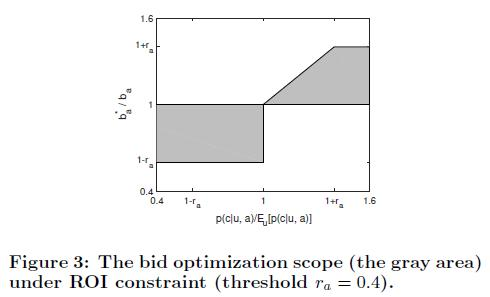### 3. Ranking

在依赖eCPM的排序机制下，在可行域内选取不同的$b_a^*$ 可能会导致排序结果的不同，进而影响到其他的指标。

先看最简单的情况，只有1个广告位，候选广告集合A中共有n个广告，通过调整出价来竞争这个广告位，优化问题的数学形式如下：
$$\max_{b_1^*,\cdots,b_n^*}f(k,b_k^*)\qquad\qquad\qquad\qquad \\ s.t.\qquad k=\mathop{\arg\max}_i\ pctr_i*b_i^* \\ \qquad\qquad l(b_i^*)\le b_i^*\le u(b_i^*),\forall i\in A$$
$k$是最终胜出的广告，依赖于$b_1^*,\cdots,b_n^*$ 的选取，$f(\cdot)$ 是需要优化的目标函数，综合了我们关注的指标。比如下面两个例子：
$$f_1(k,b_k^*)=pctr_k*pcvr_k*v_k\qquad\qquad\qquad \\ f_2(k,b_k^*)=pctr_k*pcvr_k*v_k+\alpha*pctr_k*b_k^*$$
$f_1$只考虑GMV，而$f_2$同时考虑GMV和eCPM即广告平台收入。论文后面的实验部分给出了一种更复杂的形式，同样是综合GMV和eCPM：
$$f(k,b_k^*)=pctr_k*b_k^**(1+\sigma(\frac{pcvr_k*v_k*||A||}{\sum_{i\in A}pcvr_i*v_i},w)*r_a)$$

以上所有的$f(k,b_k^*)$ 函数都是$b_k^*$ 的单调增函数（更准确的说应该是单调非减函数，论文里没有严格区分），这为后面的ranking算法提供了便利。一般来说，这个单调增的假设也是合理的，毕竟出价高了对于大部分指标都是好事。

• 优化问题求解方法：
设$s_a^*=pctr_a*b_a^*$，则$s_a^*$ 的下界和上界分别为$l(s_a^*)=pctr_a*l(b_a^*)$，$u(s_a^*)=pctr_a*u(b_a^*)$，将所有的$f(i,u(b_i^*))$ 按降序排列，然后按顺序找出第一个广告$k$，满足$u(s_k^*)$ 大于等于其他所有的$l(s_i^*)$，这个$k$就是最终展示的广告，且$b_k^*=u(b_k^*)$。

• 论文省略了求解方法正确性的严格证明，这里补充一下：
a. 首先最终结果$b_k^*$ 一定等于$u(b_k^*)$。
反证法：假设最终结果为$b_k^*$，且 $b_k^*\lt u(b_k^*)$ 。由约束可知 $pctr_k*b_k^*$ 大于等于其他所有的$pctr_i*b_i^*$，若取新的$b_k^{+}=u(b_k^*)$，则有$pctr_k*b_k^{+}>pctr_k*b_k^*$，$pctr_k*b_k^{+}$ 仍然大于等于其他所有的$pctr_i*b_i^*$，即最终胜出的广告还是$k$，但由$f(\cdot)$ 的单调递增性，$f(k,b_k^{+})>f(k,b_k^*)$，矛盾。
b. 由a可知，$f(\cdot)$ 的最大值一定是某个$f(i,u(b_i^*))$，$i$从1到$n$。我们当然希望越大越好，因此从大到小排列挨个检验，$f(k,u(b_k^*))$ 能雀屏中选的前提是$u(b_k^*)$ 实力够硬，能够在其他$b_i^*$ 配合的情况下满足约束条件：$pctr_k*u(b_k^*)$ 大于等于其他所有的$pctr_i*b_i^*$，既然其他$b_i^*$ 很配合，当然都取最小值$l(b_i^*)$ 最好，所以$u(b_k^*)$ 的条件放松到$pctr_k*u(b_k^*)$ 大于等于其他所有的$pctr_i*l(b_i^*)$，也即$u(s_k^*)$ 大于等于其他所有的$l(s_i^*)$。
证毕。
综上，$f(\cdot)$ 的单调性是算法正确性的保证，复杂度也不高，主要就是个排序。

说完1个广告位的情况，再推广到N个广告位，算法如下图，本质上是个贪心算法，先按照1个广告位的算法找出广告$k$放到广告位1，然后调整剩下广告的$u(s_i^*)\le u(s_k^*)$，不然$k$就不能保证排在第1位了，同时调整剩下广告的$u(b_i^*)$，接着在剩下的广告中按1个广告位的算法找出第2个广告位的广告，依次类推：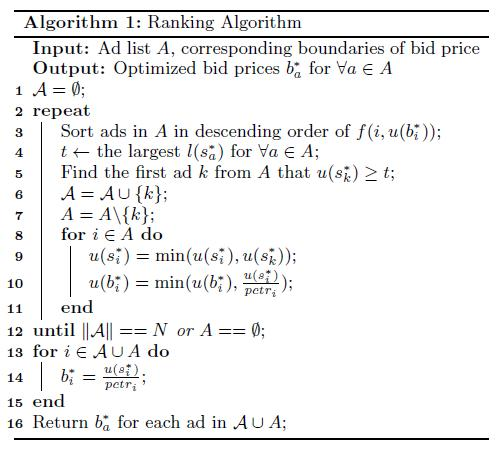### 4. 模型校正（Calibration）

论文提到他们模型的pCVR有偏差，当真实CVR越大，pCVR和真实CVR的比值越大，也即偏差越大，所以需要校正。论文根据实验观察给出了一个校正的经验公式：
$$p(c|u,a)= \begin{cases} p(c|u,a), & p(c|u,a)<tc \\ p(c|u,a)*(1+\log(\frac{p(c|u,a)}{tc})), & p(c|u,a)\ge tc \\ \end{cases}$$
$tc$为阈值，取0.012。我们之前的做法是采用保序回归，不知道孰优孰劣。

### 5. 模型评估

这篇阿里的论文也说他们碰到了类似的现象，于是想出一个新的指标Group AUC (GAUC)，即将测试数据按照用户u和广告位p的组合(u,p)分组计算AUC（如果某个组全是正样本或全是负样本，则忽略这个组），最后再按权重求平均，权重可以是各组的展示次数或点击次数。具体公式如下：
$$GAUC=\frac{\sum_{(u,p)}w_{(u,p)}*AUC_{(u,p)}}{\sum_{(u,p)}w_{(u,p)}}$$
可惜论文并没详细说这么做到底能带来多少好处，是否真的解决了前面AUC线下和线上不一致的弊端。

## 实验结果

论文分线下模拟和线上效果做了很多角度的实验对比，这里只记录几个主要的部分。

### 1. 线下模拟

通过历史的log数据，将pCTR和pCVR当做真实的CTR和CVR，比如某次展示的广告计算出pCTR为4%，则认为贡献了0.04的点击。然后设计各种策略，统计关心的指标。

• Strategy 0为对照组，保持原来线上的状态
• Strategy 1站在广告主角度，设定一个简单的调价格规则$b_a^*=b_a*(1+\sigma(\frac{p(c|u,a)}{E_u[p(c|u,a)]},w)*r_a)$
• Strategy 2就是OCPC策略，优化的目标函数前面说过，是$f(k,b_k^*)=pctr_k*b_k^**(1+\sigma(\frac{pcvr_k*v_k*||A||}{\sum_{i\in A}pcvr_i*v_i},w)*r_a)$
• Strategy 3不调出价而是直接修改rankscore公式，不再是eCPM排序，而改成按$pctr*pcvr*bid$，很明显是想提升GMV

实验结果如下图，相对Strategy 0，Strategy 1和3的GPM（即千次展示GMV）和ROI都提高了但RPM降了，只有Strategy 2即OCPC策略在3个指标上都获得了提升，达到三方共赢。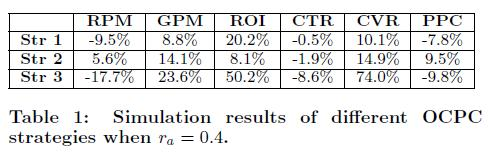### 2. 线上效果

Strategy 2相比Strategy 0和线下模拟一致，依然在三个指标上都获得了提升，见下图：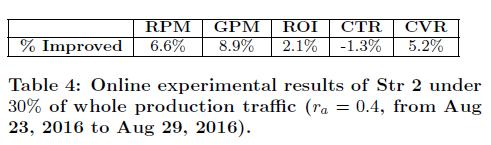论文始终强调，这套机制具有普适性，并不局限于GMV，论文给出一个例子，双十一前淘宝商家更加关注商品加入购物篮的量，于是使用模型预测的商品加入购物篮概率pASR，修改目标函数为：
$f(k,b_k^*)=pctr_k*b_k^**(1+\sigma(\frac{pasr_k*||A||}{\sum_{i\in A}pasr_i},w)*r_a)$，

## 总结

这又是一篇从实践中来的论文，针对真实场景的问题建模，算法原理也不是多么的高深复杂，却取得了很好的效果。阿里在广告主数据方面得天独厚，广告主本身就是淘宝商家，ROI的数据可以轻易获得。如果是别的广告平台想做ROI优化可能会困难重重，首先各家广告主的ROI诉求可能千差万别，其次数据共享更是难如登天。

# 论文学习：Explore and Exploit

## 前言

前阵子读了一篇KDD2017的论文”A Practical Exploration System for Search Advertising”，是有关E&E算法的，放假无事便调研了一些相关资料，做个小结。

计算广告和推荐系统中经常会碰到某些长尾广告或长尾item从来没有机会或者很少机会的展示，导致CTR预估非常不准，需要探索性地创造机会给它们一定的展示量，但又不能带来太大的损失。这种问题一般称作Explore and Exploit问题。

在学术界经常把它描述成为一个多臂赌博机问题(multi-armed bandit problem, MAB)，若干台赌博机，每次可以选择一台机器摇一下，有一定概率会吐钱出来，但各台机器吐钱概率不一致且未知，那么赌徒每次该如何选择来最大化收益？对于K-armed bandit problem数学定义为：

$i$ 为机器下标，$1\le i\le K$ ，每台机器对应一组随机变量$X_{i,1},X_{i,2},X_{i,3}…$，表示每次被选中后的收益，这组随机变量独立同分布，期望 $\mu_i$未知。

策略A是一个算法，每次选择一台机器获得其收益，定义$T_i(n)$ 为前n次选择中机器$i$被选中的次数，定义策略A的前n次选择的regret为：
$$\mu^*n-\mu_j\sum_{j=1}^{K}E[T_j(n)]$$
其中
$$\mu^*\mathop{=}^{def}\max_{1\le i\le K}\mu_i$$
regret越小表示策略A越好，看起来有点像online learning的regret定义。下面总结几种最近调研的E&E算法。

最简单的办法，每次随机选。

## 二：最大均值

先随机选若干次，然后一直选均值最大的。

## 三：$\epsilon$-Greedy

设定参数 $\epsilon\in(0,1]$，每次以 $\epsilon$ 的概率随机选一个机器，否则选择当前收益均值最大的机器。

论文中给出了一个变种算法：$\epsilon_n$-Greedy，具体为 $\epsilon$ 不再固定，而是以1/n的速率衰减，可以证明比原始方法有更好的regret。具体见论文的Theorem 3。

## 四：Thompson Sampling

对于收益为1和0二值的情况，可以假定每台机器$i$收益符合参数为$p_i$的伯努利分布，假定参数 $p_i\sim Beta(\alpha_i,\beta_i)$，（搞过LDA的应该都知道Beta分布和伯努利分布的共轭关系）。

算法具体为：每一轮每台机器用其当前自身的Beta分布生成一个数$p$，本轮选择$p$最大的那台机器，假定下标为$i$；然后观察其收益，如果为1则 $\alpha_i$加1，为0则 $\beta_i$加1。

## 五：UCB(Upper Confidence Bound)

论文中不止一个UCB，这里只介绍最简单的UCB1。

1. 初始化：每台机器选择一次

2. 循环：选择机器$i$，满足
$$i=\mathop{\arg\max}_{1\le j\le K}(\bar x_j+\sqrt{\frac{2\ln n}{n_j}})$$
其中，$\bar x_j$是机器$j$的平均收益，$n_j$是机器$j$被选中的次数，$n$为总的轮次数。

算法思想很明白，历史均值加上一个置信区间来估计本轮的收益，历史被选中的次数越少则置信区间越大，会加大被选中的机会。

可以证明，UCB的regret量级为O(log n)，具体证明过程见论文，证明过程比较复杂，我看了很久才看明白，会用到Chernoff-Hoeffding bound不等式和1/n^2的无穷级数求和。

## 六：LinUCB

LinUCB算法是雅虎2010年提出的，用于新闻推荐，见论文。考虑到前面几种算法都过于简单，根本没有考虑到个性化的问题，不同的user对于同样的item的期望收益（比如CTR）也是不一样的，且item本身也可能随时间动态变化，因此论文重新定义了考虑上下文的MAB问题，在第t轮：

1. 算法A观察到当前用户$u_t$和当前的item候选集$A_t$，对于每一个$a\in A_t$和当前$u_t$，有一个特征向量$x_{t,a}$，即代表上下文

2. 通过前t-1轮的收益结果，算法A选择一个$a_t\in A_t$，然后得到收益$r_{t,a_t}$，这里 $r_{t,a_t}$ 的期望与$u_t$和$a_t$都有关

3. 根据新的观测值 $(x_{t,a_t},a_t,r_{t,a_t})$，算法A更新选择策略

论文中给出两种LinUCB，我们这里只说相对简单的第一种。

LinUCB假定$r_{t,a}$ 的期望值和 $x_{t,a}$ 成线性关系，这也是LinUCB名字的来历，具体为：
$$E[r_{t,a}|x_{t,a}]=x_{t,a}^T\theta_a^*$$
可以看到每一个item $a$对应一个未知参数 $\theta_a^*$，可以通过历史数据来估计。假定在第t轮的时候，$a$被选中过m次，对应的特征向量和收益构成训练数据 $(D_a,c_a)$，$D_a$为$m\times d$矩阵，即m个特征向量，$c_a$为$m\times 1$向量，即m个收益。

通过岭回归可以得到 $\theta_a^*$ 的估计值：
$$\hat\theta_a=(D_a^TD_a+I_d)^{-1}D_a^Tc_a$$

### 备注-岭回归的推导：

对于线性回归$D\theta=c$，采用最小二乘和L2正则项来估计参数 $\theta$.
$$\min_\theta f(\theta)=\min_\theta||c-D\theta||_2^2+\lambda||\theta||_2^2\\ f(\theta)=(c-D\theta)^T(c-D\theta)+\lambda\theta^T\theta\\ \Rightarrow df=(d(c-D\theta))^T(c-D\theta)+(c-D\theta)^Td(c-D\theta)+\lambda(d\theta)^T\theta+\lambda\theta^Td\theta\\ =2(c-D\theta)^Td(c-D\theta)+2\lambda\theta^Td\theta\\ =-2(c-D\theta)^TDd\theta+2\lambda\theta^Td\theta$$
令$df=0$，有
$$D^T(c-D\theta)=\lambda\theta\\ \Rightarrow D^Tc=(D^TD+\lambda I)\theta\\ \Rightarrow \theta=(D^TD+\lambda I)^{-1}D^Tc$$

回到正题，得到 $\hat\theta_a$后，有：

对于任意 $\delta>0,\alpha=1+\sqrt{\ln(2/\delta)/2}$，
$$P\left\{\left|x_{t,a}^T\hat\theta_a-E[r_{t,a}|x_{t,a}]\right|\le\alpha\sqrt{x_{t,a}^T(D_a^TD_a+I_d)^{-1}x_{t,a}}\right\}\ge1-\delta$$
这部分的推导要用到论文的定理，我还没来得及细看。

有了这个置信区间的不等式，就可以类似UCB算法一样，在第t轮选择：
$$a_t\mathop{=}^{def}\mathop{\arg\max}_{a\in A_t}\left(x_{t,a}^T\hat\theta_a+\alpha\sqrt{x_{t,a}^T(D_a^TD_a+I_d)^{-1}x_{t,a}}\right)$$
算法具体如下图：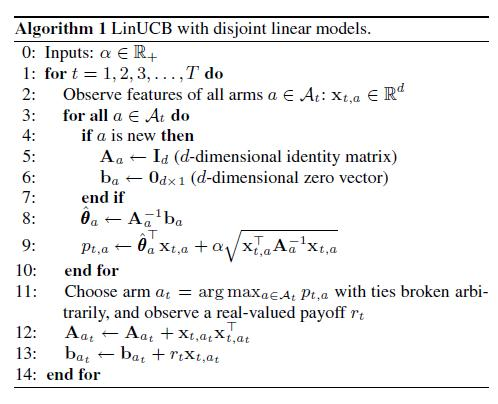可以看到，算法涉及矩阵求逆等运算，如果特征向量维度很高，计算会很复杂。因此论文介绍了如何构建特征，主要是如何降维。user相关的原始特征1193维，item相关的原始特征83维，user特征用 $\phi_u$ 表示，item特征用 $\phi_a$ 表示。用LR构建点击模型来拟合历史数据，不过线性部分比较特别，形式为 $\phi_u^TW\phi_a$，即考虑了user和item的所有二阶特征组合，通过LR训练出参数矩阵$W$后，将user特征投影到item的特征空间：
$$\psi_u\mathop{=}^{def}\phi_u^TW$$
然后再聚类为5个簇，再加上偏置项，即特征向量 $x_{t,a}$ 一共只有6维，计算将大大简化。

论文还有一个有意思的部分，介绍了如何offline来评估E&E算法的好坏。比如我们有一份完全随机策略的log数据，希望能够线下评估我们新的E&E算法A，具体为：

1. 依次扫描log数据的每一条记录，如果展示的item和算法A给出的不一致，则丢弃该条记录，直到找到和算法A一致的一条记录

2. 该记录加入到算法A的历史，算法A更新选择策略

3. 该记录的收益加入到A的总收益，如果A的历史记录不够T条，回到第1步，从上次的位置继续扫描

4. 直到A的历史数据达到T条，最后输出总收益除以T

## 七：Exploration Algorithm for Search Ads

前面的算法都是把E&E独立出来讲，假如我们的业务已经有了一个click model，那该如何和E&E结合呢？A Practical Exploration System for Search Advertising给出了一个很好的方案。这篇论文来自KDD2017，是雅虎搜索广告团队的工作。

冷启动问题特化到计算广告领域，就是指广告主向广告系统提交新的广告，系统很难准确预估新广告的CTR，带来的后果是新的广告可能拿不到多少展示量，click model不能很快学习到这些“冷广告”的点击概率，影响整体效果（比如收入）。

仔细分析，如果对新广告的CTR预估高了还好一些，初期就会有足够的展示量，只要模型更新及时，很快学习到更加准确的点击率，然后恢复正常；如果新广告的CTR预估很低，拿不到展示量，模型不能学到准确点击率，即使模型更新，预估还是偏低，恶性循环，导致本来质量不错的新广告永远拿不到量。

因此这篇论文的思想便是对CTR预估较低的新广告做boosting，加大预估CTR，具体实现结合了 $\epsilon$-Greedy和UCB的思路。

### 一些背景知识：

搜索广告被Matching模块召回，但拿不到展示量的原因主要有：

2. Reserve Price：出于商业利益，广告系统一般会设定一个最低价格的限制，如果bid*pCTR低于这个最低价格，则连参与竞价的机会都没有。

3. Auction：最后竞价环节，狼多肉少，广告坑位有限，大家按rank-score排序，分高者胜出。而bid*pCTR又是rank-score的最重要组成部分。

显然，这人生的三道坎都与预估CTR直接相关，可见click model何等重要。

click model干的事就是给定搜索词q、广告a、用户u之后，预估u对a的点击概率p(click|q,a,u)，一般都采用基于特征的监督学习模型。而最重要的特征往往是所谓的点击反馈特征，即历史点击数和历史展示数以及二者的比值历史CTR，如果考虑了位置偏置，就变成EC/COEC特征。

EC/COEC在多个雅虎的论文里提到过，不过最早的出处应该是2007年的这篇。简单来说，搜索广告位有多个，比如竖排一列，不同rank的点击率天然就有差异，一般高处rank的偏高，低处rank的偏低，这就是位置偏置。这种天然点击率可以通过统计每一个rank上的所有点击除以所有展示来得到，当然这是一种近似值，因为广告系统会把rank-sore高的广告往高rank处放，这样统计又引入了rank-score偏置，除非开一部分流量完全随机出广告。

我们将每个rank上的天然点击率记为 $ctr(r)$，r为rank编号。比如 $ctr(r_1)$ 是 $ctr(r_2)$ 的两倍，广告A在$r_1$上展示了1000次，点击了100次，广告B在$r_2$上展示了1000次，点击了80次，如果直接将这些数字作为特征的话，模型可能会学出广告A的点击率大于广告B，但其实考虑位置偏置，广告A的点击率小于B才是合理的。

为此定义expected clicks(EC)为某个广告a在rank r上的期望点击数：
$$ec(r,a)=ctr(r)*i_r(a)$$

定义clicks over expected clicks(COEC)如下：
$$COEC(a)=\frac{\sum_r c(r,a)}{\sum_r ec(r,a)}$$
其中 $c(r,a)$ 为a在rank r上的实际点击数。

$EC(a)=\sum_r ec(r,a)$ 可以看做是广告a历史展示数的一种normalization，如果非要在绝对值上较真的话可以除以最大的 $ctr(r)$，变成
$$EC(a)/\max_r ctr(r)，$$

同理，COEC(a)可以看做是对广告a的历史CTR做normalize，去除位置偏置。

论文算法具体如下图，该算法应放在click model输出预估CTR之后、auction模块之前：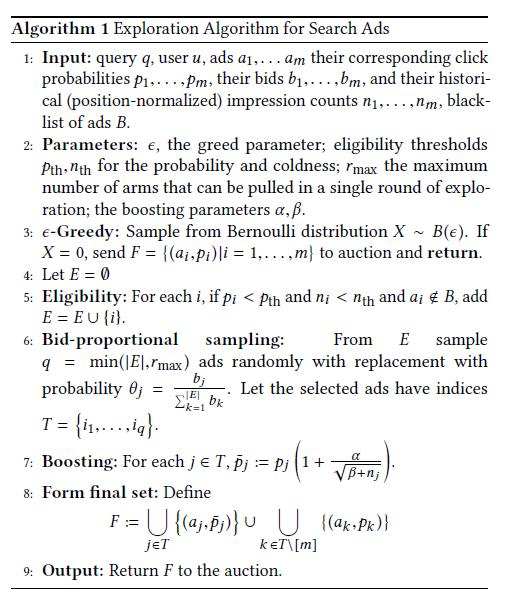1：输入，包括当前搜索词q，用户u，召回的广告列表，相应的pCTR，出价bids，和每个广告的历史展示数（去除了位置偏置）。还贴心地准备了一个黑名单列表，即在黑名单里的新广告就不要指望boosting了，听天由命吧，应该是防止严重影响用户体验的广告得到boosting。

2：参数，下面碰到了细说。

3：照搬 $\epsilon$-Greedy的思路，只在小流量上做Exploration，论文给出的参数 $\epsilon=0.05$，还是比较保守的。

4：设定一个要参与Exploration的广告候选集E，先置为空集。

5：构建候选集E，对所有召回的广告，如果pCTR小于阈值 $p_{th}$ 且历史展示数小于阈值 $n_{th}$ 且不在黑名单的，加入E。即只对足够新的、预估CTR偏低的广告做Exploration。论文给出的参数 $n_{th}=500$，$p_{th}$ 和AQF模块的阈值一致，为0.02。

6：E集合还是偏大，做不到人人有份，所以要抽签决定最终参与boosting的广告，最多 $r_{max}$ 个，论文给出的 $r_{max}$ 只有2。这里抽签的方法要细讲一下，因为论文是把这个作为一个创新点的。抽签采用轮盘赌的方式而不是纯随机，bid越大，被选中的概率越大。这样做的好处有三，一是选出bid高的，最终在auction环节胜出的几率也大，否则折腾半天都白费功夫了；二是保证整体的price-per-click(PPC)够高，防范Exploration带来收入上的损失；三是给了广告主一个正反馈，广告主经常抱怨我在你们这投了新广告钱出的老高怎么量不见涨啊，这下好了，给钱好办事，你出价高我给你量就多，一定程度上还能刺激收入。

7：最重要的boosting，将pCTR变大，仿照的是UCB的思路，即在原来pCTR的基础上加上个置信区间。这里的置信区间给的有些任性，论文似乎想从伯努利分布的标准差推导出来，但很不严谨。不管了，反正还有两个参数要调，$\beta$ 是防止分母为0，$\alpha$ 要好好调调，保证boosting之后的广告能有80%越过AQF那道坎。

8：形成最终集合F，经过boosting的广告和剩下的广告合并。这里公式似乎有误，$k\in T\setminus[m]$ 应该是 $k\in [m]\setminus T$。

9：把最终集合F送到auction模块。

算法评估：

1. Learning Rate Metrics：论文定义了一个函数，评估新广告从冷变热的速度，实验证明相比对照组，引入boosting后确实变快了。意料之中，无需细说。

整篇论文给人的感觉就是很“工程”，没有繁琐的理论推导，而有详细的算法框架，原理也很简单直接，还给出了具体参数，最后再给出实际线上效果让你放心。总之就是对我等工程师很友好，拿来即可用，稍微改改就能用到搜索、推荐等领域。

 Finite-time Analysis of the Multiarmed Bandit Problem, 2002
 A Contextual-Bandit Approach to Personalized News Article Recommendation, 2010
 Exploring compact reinforcement-learning representations with linear regression, 2009
 A Practical Exploration System for Search Advertising, 2017
 Comparing Click Logs and Editorial Labels for Training Query Rewriting, 2007

# lambdaFM

## 前言

前阵子github上有人问我能不能实现一下基于FM的排序模型，于是周末就在alphaFM基础上修改一番，类似lambdaMART的思路，把lambdaRank和FM结合，实现了lambdaFM。

代码地址以及使用方法在：
https://github.com/CastellanZhang/lambdaFM

同样是FTRL的online learning优化方法，支持高维稀疏特征，单机多线程版本。

lambdaFM同时也实现了pairwise的算法，即不考虑deltaNDCG，可以通过参数-rank来选择使用lambdaRank还是pairwise。

## pairwise

让我们从pairwise说起，以搜索为例，对于一对样本 $\langle x^i,x^j\rangle$ ，如果 $x^i$ 的相关性好于 $x^j$，我们记为 $x^i\triangleright x^j$ ,反之为 $x^i\triangleleft x^j$ ，相应目标值 $y_{ij}$ 为1或0。建立概率模型如下：
$$P_{ij}=P(x^i\triangleright x^j|\Theta)=P(y_{ij}=1|\langle x^i,x^j\rangle,\Theta)\\ =\sigma(\hat{y}(x^i|\Theta)-\hat{y}(x^j|\Theta))=\frac{1}{1+e^{-(\hat{y}(x^i|\Theta)-\hat{y}(x^j|\Theta))}}$$
其中 $\hat{y}(x|\Theta)$ 就是FM的输出：
$$\hat{y}(x|\Theta):=w_0+\sum_{i=1}^nw_ix_i+\sum_{i=1}^n\sum_{j=i+1}^n\langle v_i,v_j\rangle x_ix_j\\ =w_0+\sum_{i=1}^nw_ix_i+\sum_{i=1}^n\sum_{j=i+1}^nx_ix_j\sum_{f=1}^kv_{i,f}v_{j,f}\\ =w_0+\sum_{i=1}^nw_ix_i+\frac{1}{2}\sum_{f=1}^k\left(\left(\sum_{i=1}^nv_{i,f}x_i\right)^2-\sum_{i=1}^nv_{i,f}^2x_i^2\right)$$
模型参数估计仍然用最大似然，对于所有训练样本对集合 $S$ ，最优化问题为：
$$\mathop{\arg\max}_{\Theta}\prod_{(\langle x^i,x^j\rangle,y_{ij})\in S}P_{ij}^{y_{ij}}(1-P_{ij})^{1-y_{ij}}$$
对于训练样本集合 $S$ ，我们总可以调整每一对样本的顺序使得总是有 $x^i\triangleright x^j$ ，即所有的 $y_{ij}$ 都等于1，上面公式简化为：
$$\mathop{\arg\max}_{\Theta}\prod_{(\langle x^i,x^j\rangle,1)\in S}P_{ij}=\mathop{\arg\min}_{\Theta}\sum_{(\langle x^i,x^j\rangle,1)\in S}-\ln P_{ij}$$
这样每一对样本 $\langle x^i,x^j\rangle$ 的损失函数为：
$$l(\Theta|\langle x^i,x^j\rangle)=-\ln P_{ij}=\ln (1+e^{-(\hat{y}(x^i|\Theta)-\hat{y}(x^j|\Theta))})$$
损失函数对参数求偏导数：
$$\frac{\partial l}{\partial\theta}= \frac{\partial l}{\partial(\hat{y}(x^i|\Theta)-\hat{y}(x^j|\Theta))}(\frac{\partial\hat{y}(x^i|\Theta)}{\partial\theta}-\frac{\partial\hat{y}(x^j|\Theta)}{\partial\theta})$$
我们令：
$$\lambda_{ij}=\frac{\partial l}{\partial(\hat{y}(x^i|\Theta)-\hat{y}(x^j|\Theta))}=-\frac{1}{1+e^{\hat{y}(x^i|\Theta)-\hat{y}(x^j|\Theta)}}$$
则上面公式可以简化为：
$$\frac{\partial l}{\partial\theta}= \lambda_{ij}(\frac{\partial\hat{y}(x^i|\Theta)}{\partial\theta}-\frac{\partial\hat{y}(x^j|\Theta)}{\partial\theta})$$
而FM的输出对参数的偏导数在alphaFM的介绍中已经给出过，下面直接列出。注意，由于是pair的方法，偏置项 $w_0$ 相减总会抵消掉，我们可以固定 $w_0$ 为0，不需要再求解，也就不需要对 $w_0$ 再算偏导数：
$$\frac{\partial\hat{y}}{\partial\theta}= \begin{cases} x_i, & if\,\,\theta\,\,is\,\,w_i \\ x_i\sum_{j=1}^nv_{j,f}x_j-v_{i,f}x_i^2 & if\,\,\theta\,\,is\,\,v_{i,f} \\ \end{cases}$$
有了损失函数对参数的偏导，后面优化就是水到渠成。

## lambdaRank

在上面的pairwise方法中，可以看到对于每一对样本都是同等对待的，算法尽量使得每一对样本的label大小关系都能预测对，而对于它们具体的label是多少以及在展现中的位置并不敏感。但在实际问题中，当评价指标是NDCG等时，这些信息就很重要了。举个例子：比如同一query下召回了4个doc，实际相关性分数分别为0 1 3 4，我们有两个排序模型A和B，通过模型的打分，排列结果分别为4 3 0 1和3 4 1 0。从pairwise的角度来看，两个排列跟最优排列4 3 1 0都只相差一次交换，似乎模型效果没差别，但是从NDCG的角度来看，NDCG(A) = 0.997，NDCG(B) = 0.852，明显模型A的效果更好。

为了迎合NDCG指标，需要在训练的时候对样本pair区别对待，设置不同的权重，lambdaRank提出了一种权重deltaNDCG，即在原来的顺序上如果交换样本 $i$ 和样本 $j$ ，带来的NDCG值变化的绝对值。形式上是将上面公式的 $\lambda_{ij}$ 乘以权重系数，变成 $\lambda_{ij}’$ ：
$$\lambda_{ij}’=\lambda_{ij}|\Delta NDCG_{ij}|$$
这应该就是lambda名字的来源。在我看来，lambdaRank其实仍然是一种pairwise的方法，因为并没有直接对list做优化，跟ListNet等listwise方法有本质不同。

# MLR, PLM

## 前言

最近阿里的盖坤大神放出了一篇论文Learning Piece-wise Linear Models from Large Scale Data for Ad Click Prediction，介绍了阿里广告的一个主要ctr预估模型Large Scale Piece-wise Linear Model (LS-PLM)，在2012年就开始使用，据说早期叫做Mixture of LR(MLR)。

看完论文就很想验证一下效果，于是基于原来的alphaFM代码很快就实现了一个单机多线程版本，优化算法用了FTRL。完成代码的时候正好又赶上alphaGo完虐人类，于是命名仍然冠以alpha，叫做alphaPLM。

## 算法原理

PLM可以看做是混合了聚类和分类的思想，即将特征空间分片或者说分区间，每个分片就是一个聚类，每个聚类对应一个单独的线性模型LR。这里的聚类是软聚类，即每个样本可以属于多个分片，有概率分布。最后计算ctr是先算出在每个分片的ctr，再按属于各个分片的概率加权平均。通过分片线性拟合，达到了非线性的效果。

具体模型公式如下：

$$p(y=1|x)=\sum_{i=1}^{m}\frac{e^{u_i^Tx}}{\sum_{j=1}^me^{u_j^Tx}}\cdot\frac{1}{1+e^{-w_i^Tx}}\\ =\sum_{i=1}^{m}\frac{e^{u_i^Tx}}{\sum_{j=1}^me^{u_j^Tx}}\cdot\sigma(w_i^Tx)$$

可以看到，聚类部分是用了softmax函数，分类部分就是LR的sigmoid函数。该算法的巧妙之处就是将二者合成一个公式，一起训练参数。公式中m是分片数，属于超参数，由人工给定。模型参数是 $\Theta=\{u_1,…,u_m,w_1,…,w_m\}\in R^{d\times 2m}$，需要训练得到。

论文中的优化方法采用的是LBFGS，实现起来比较复杂，我为了快速验证算法效果，优化改成了FTRL，需要计算损失函数对u和w的梯度，下面给出推导。

首先对于 $y\in\{-1,1\}$，模型可以统一形式：

$$p(y|x)=\sum_{i=1}^{m}\frac{e^{u_i^Tx}}{\sum_{j=1}^me^{u_j^Tx}}\cdot\frac{1}{1+e^{-yw_i^Tx}}\\ =\sum_{i=1}^{m}\frac{e^{u_i^Tx}}{\sum_{j=1}^me^{u_j^Tx}}\cdot\sigma(yw_i^Tx)$$

单条样本(x,y)的损失函数：
$$l(\Theta|x,y)=-\ln P(y|x,\Theta)=-\ln\frac{1}{\sum_{j=1}^me^{u_j^Tx}}\sum_{i=1}^{m}e^{u_i^Tx}\sigma(yw_i^Tx)\\ =\ln\sum_{j=1}^me^{u_j^Tx}-\ln(\sum_{i=1}^{m}e^{u_i^Tx}\sigma(yw_i^Tx))$$
梯度计算：
$$\nabla_{u_k}l=\frac{e^{u_k^Tx}x}{\sum_{j=1}^me^{u_j^Tx}}-\frac{e^{u_k^Tx}\sigma(yw_k^Tx)x}{\sum_{i=1}^{m}e^{u_i^Tx}\sigma(yw_i^Tx)}$$
$$\nabla_{w_k}l=\frac{ye^{u_k^Tx}\sigma(yw_k^Tx)(\sigma(yw_k^Tx)-1)x}{\sum_{i=1}^{m}e^{u_i^Tx}\sigma(yw_i^Tx)}$$
后续的FTRL算法框架和alphaFM非常类似，不再详述，可以参见之前的文档和实现代码。

## 算法效果

论文中给了一个demo数据的例子，如下图：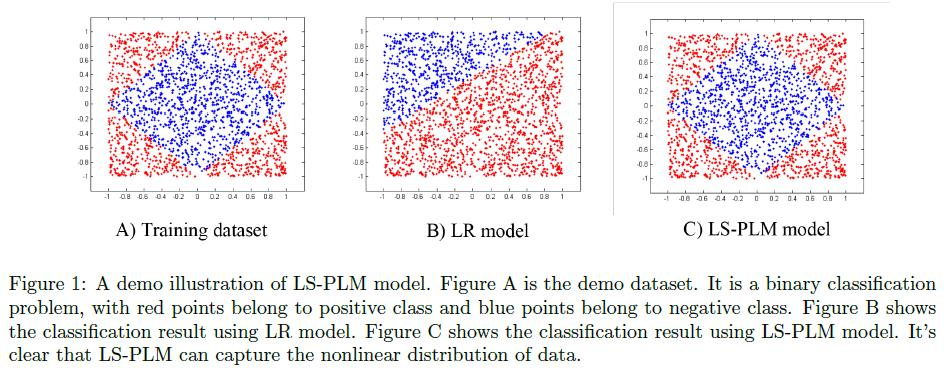我们可以通过代码生成类似的样本，来验证一下算法效果：

生成1万条训练样本和2000条测试样本：

python demo_data1.py 10000 > train.txt

python demo_data1.py 2000 > test.txt

alphaPLM的训练参数如下：

cat train.txt | ./plm_train -m model.txt -u_bias 1 -w_bias 1 -u_l1 0.001 -u_l2 0.1 -w_l1 0.001 -w_l2 0.1 -core 1 -piece_num 4 -u_stdev 1 -w_stdev 1 -u_alpha 10 -w_alpha 10

在测试集的AUC可以达到0.99以上。

如果是LR或FM，你会发现无论你怎么调参，AUC始终在0.5左右。

直观上也很容易理解，看图就会发现，如果特征就是x和y的坐标值的话，数据非线性可分，而很明显在四个象限的分片里分别都是线性可分的。

你心里是否已经忍不住开始唾弃LR：“啊呸，LR果然是个战五渣！连这么个demo数据都搞不定！”那你可就冤枉LR了，原始特征非线性，完全可以通过转换变成线性或近似线性，比如做个简单的离散化就会大不一样：

重新生成1万条训练样本和2000条测试样本：

python demo_data2.py 10000 > train.txt

python demo_data2.py 2000 > test.txt

此时你会发现，无论LR、FM还是PLM，AUC都很容易达到0.99以上。

而在我们广告业务的真实数据中，LR所面对的几乎都是千万维或上亿维的高维离散特征，效果并不会比FM或PLM差太多。我在真实数据的实验也验证了这一点，PLM和FM相比LR提升的都差不多，AUC基本都是在千分位提高几个点。

# Ensembling, Lagrange

据我观察，名字带“拉”的人一般都很厉害，比如马拉多纳、希拉里、狄波拉、张娜拉、杜拉拉、陈法拉、尼古拉斯·赵四、地铁站的罗拉……

但跟我们今天的大神拉格朗日（Lagrange）一比就都被秒成了渣渣，因为每一个上过高数和物理的我们都被拉大神无情碾压摩擦过啊~

童鞋，请站起来回答拉格朗日中值定律是啥？

童鞋，请站起来回答拉格朗日乘子法是啥？

童鞋，请站起来回答拉格朗日内插公式是啥？

童鞋，请站起来回答拉格朗日点是啥？

童鞋，请站起来回答拉格朗日函数是啥？

童鞋，请站起来回答拉格朗日方程是啥？

……

童鞋，你肿么站不起来了童鞋？

请原谅我召唤出封印在你们心底多年的梦魇，快跟我一起踏碎时空大逃亡，继续闪回到小米数据挖掘大赛那几天。

上文中已经提到我们最终的模型不止一个，而是多个DNN和FM共七八个模型的融合。

所谓模型融合，洋气一点叫Ensembling，在 http://mlwave.com/kaggle-ensembling-guide/ 一文中有详细介绍。简单来说就是综合多个单模型的输出来给出最终的结果，一般会比每个单模型的效果都好，现在已经是各大比赛的常规武器。但对于初涉江湖的小伙伴们还没有太多经验，一开始只是简单的平均，后来尝试了各个模型的输出做为LR的特征，发现没啥卵用，比赛已近尾声就没再折腾更复杂的方法，最终使用线性加权平均，即：
$$\hat y=w_1\hat y_1+w_2\hat y_2+…+w_M\hat y_M$$
其中，$w_1+w_2+…+w_M=1$

这里有个问题就是权重系数怎么定？

一开始就是拍脑袋定，单模型效果好的权重就大一点，效果差的就小一点。后来小伙伴使用暴力网格搜索的方法，融合两三个模型还行，再多就已经慢到不可接受。

我看在眼里急在心头，我们是模武双修的种族啊，怎么能只用暴力解决呢？这种目标如此鲜明灿若煌煌皓月的好问题，当然要祭出流光华丽的大模型才相得益彰呢！

## 第一式：混沌初分模型现！

比如我们有M个单模型分类器，解决K分类问题，测试集包含N条样本，$\hat{y}_{nmk}$ 表示第m个单模型对第n条样本属于第k类的预测概率。

我们采用线性加权平均的方法，融合M个模型得到的预测概率
$$\hat{y}_{nk}=\sum_{m=1}^{M-1}w_m\hat{y}_{nmk}+(1-\sum_{m=1}^{M-1}w_m)\hat{y}_{nMk}$$
比赛评价标准为logloss，我们希望融合后的模型在测试集上的logloss尽量小，所以优化目标如下：
$$\min_{w}f(w)=\min_{w}\{-\frac{1}{N}\sum_{n=1}^{N}\sum_{k=1}^{K}1\{y_n=k\}\ln\hat{y}_{nk}\}\\ =\min_{w}\{-\frac{1}{N}\sum_{n=1}^N\sum_{k=1}^{K}1\{y_n=k\}\ln(\sum_{m=1}^{M-1}w_m\hat{y}_{nmk}+(1-\sum_{m=1}^{M-1}w_m)\hat{y}_{nMk})\}$$
其中$w=[w_1,..,w_{M-1}]^T$是需要求解的权重参数，我们知道权重之和要归一，所以不需要$w_M$这一变量，用$1-\sum_{m=1}^{M-1}w_m$代替即可。

问题已明确，下面来求解。

## 第二式：梯度杀！！

$$l_n(w)=-\sum_{k=1}^{K}1\{y_n=k\}\ln(\sum_{m=1}^{M-1}w_m\hat{y}_{nmk}+(1-\sum_{m=1}^{M-1}w_m)\hat{y}_{nMk})$$
梯度为
$$\frac{\partial l_n}{\partial w_m}=-\sum_{k=1}^{K}1\{y_n=k\}\frac{\hat{y}_{nmk}-\hat{y}_{nMk}}{\hat{y}_{nk}},\quad m=1,…,M-1$$

输入数据的格式如下：

label score11,score12,...,score1K score21,score22,...,score2K ... scoreM1,scoreM2,...,scoreMK

举个例子，比如二分类4个模型的数据片段如下：

0 0.912427,0.0875725 0.905673,0.0943273 0.911398,0.088602 0.933166,0.066834
0 0.86061,0.139389 0.865925,0.134075 0.881552,0.118448 0.872196,0.127804
1 0.364299,0.635701 0.32974,0.67026 0.323839,0.676161 0.341047,0.658953
0 0.715563,0.284437 0.713995,0.286005 0.713599,0.286401 0.713383,0.286617
0 0.948186,0.0518137 0.925587,0.0744127 0.929451,0.0705489 0.939952,0.0600485
0 0.58531,0.41469 0.588321,0.411679 0.520456,0.479544 0.671846,0.328154
1 0.0154455,0.984554 0.0150741,0.984926 0.0118866,0.988113 0.0109413,0.989059
1 0.0472074,0.952793 0.0612501,0.93875 0.065446,0.934554 0.061947,0.938053
1 0.430228,0.569772 0.366636,0.633364 0.337206,0.662794 0.5487,0.4513
0 0.97958,0.0204195 0.980348,0.0196517 0.979461,0.0205394 0.985608,0.0143915


我们的测试数据共200多万条，时间复杂度跟单模型数M呈线性关系，跑一次也就分分钟的事。为了验证算法，做了对比，对于3模型融合跑出的结果和暴力求解的结果一致。

问题似乎就这么完美解决了，但人生就像直流充电桩，上一秒还是70A的电流，下一秒就可能跳电。当我们融合更多模型时问题出现了，有的权重算出了负值！就像下面这样：

0.120181463777    gender_cut_ftrl8000_val
0.0929962960391   gender_cut_ftrl_val
0.0245135332374   gender_nn_1005_val
0.269705618425    gender_cut_ftrl2000_val
0.422565675064    gender_cut_ftrl1000_val
0.233316720486    gender_newFM_val
0.0228310140994   gender_xxFM_val
-0.186110321128   gender_result_fm


## 第三式：生死符！！！

“这生死符一发作，一日厉害一日，奇痒剧痛递加九九八十一日，然后逐步减退，八十一日之后，又再递增，如此周而复始，永无休止。每年我派人巡行各洞各岛，赐以镇痛止痒之药，这生死符一年之内便可不发。”

好啦，不吓你啦，这是金庸老先生笔下天山童姥的生死符。我这里的生死符简单得很，顾名思义，由权重符号决定单模型生死，正的留，负的弃，留下的单模型重新计算权重，有可能又有新的负值出现，再次使用生死符，直到剩下的单模型权重全部大于等于0。

还以上面那次融合为例，最终把gender_xxFM_val和gender_result_fm都干掉了，剩下的权重为：

0.122788741689    gender_cut_ftrl8000_val
0.0782434412719   gender_cut_ftrl_val
0.0331138038226   gender_nn_1005_val
0.24358375583     gender_cut_ftrl2000_val
0.446465338463    gender_cut_ftrl1000_val
0.0758049189234   gender_newFM_val


在测试集上的logloss = 0.435116，而其中单模型最好的logloss是0.436593。

相应地，这批融合在7分类问题age上效果更加明显，从最好的单模型1.35416降到1.35061。

以上便是比赛期间我们使用的全部招式。

憋走，故事还没结束，忘了塞纳河畔的拉格朗日了吗？

像我这等追求卓越的好青年岂能够留下不完美的话柄，虽然比赛结束了，我还是要把它彻底解决掉——快使出

## 第四式：铁锁横江！！！！

分析上面的模型，出现负值，是因为少了对w的非负约束，那就加上：
$$\min_{w}f(w)=\min_{w}\{-\frac{1}{N}\sum_{n=1}^N\sum_{k=1}^{K}1\{y_n=k\}\ln\hat{y}_{nk}\}\\ =\min_{w}\{-\frac{1}{N}\sum_{n=1}^N\sum_{k=1}^{K}1\{y_n=k\}\ln(\sum_{m=1}^{M-1}w_m\hat{y}_{nmk}+(1-\sum_{m=1}^{M-1}w_m)\hat{y}_{nMk})\}$$
$$s.t.\quad w_m\ge 0,\quad m=1,2,…,M-1\\ 1-\sum_{m=1}^{M-1}w_m\ge 0\qquad\qquad$$
这就成了带约束的优化问题，该怎么解呢？是时候请出拉格朗日大神了！大神蜜汁微笑，抛出一套对偶宝典，将带约束的极小化问题改造成极大极小问题，正是

## 第五式：沧海一粟！！！！！

我们先把问题标准化，假定$f(x)$，$c_i(x)$，$h_j(x)$ 是定义在$R^n$上的连续可微函数，将下面约束最优化问题
$$\min_{x}f(x)\\ s.t.\quad c_i(x)\le 0,\quad i=1,2,…,k\\ \quad \quad h_j(x)=0,\quad j=1,2,…,l$$

然后引入广义拉格朗日函数
$$L(x,\alpha,\beta)=f(x)+\sum_{i=1}^{k}\alpha_{i}c_i(x)+\sum_{j=1}^{l}\beta_{j}h_j(x)$$
其中 $\alpha_i$ 和 $\beta_j$ 是拉格朗日乘子，$\alpha_i\ge 0$。然后经过一番推来导去眉来眼去，可以证明
$$\min_x\max_{\alpha,\beta:\alpha_i\ge 0}L(x,\alpha,\beta)$$

又有，当$f(x)$，$c_i(x)$，$h_j(x)$ 等满足一定条件时
$$\max_{\alpha,\beta:\alpha_i\ge 0}\min_xL(x,\alpha,\beta)$$

$$\max_{\alpha,\beta}\theta_D(\alpha,\beta)=\max_{\alpha,\beta}\min_xL(x,\alpha,\beta)\\ s.t.\qquad \alpha_i\ge 0,\quad i=1,2,…,k$$

这一部分的具体细节懒得写了，估计你们也看不下去，真要有兴趣可以去看书，比如《凸优化》或者李航老师的《统计学习方法》，我上面的公式基本就是抄他的，但请注意书上附录C的KKT条件有误，(C.22)式和(C.23)式不应该包含在里面。

好啦，照此框架，来解决我们的问题：
$$\max_{\alpha,\alpha_m\ge 0}\min_wL(w,\alpha)\\ =\max_{\alpha,\alpha_m\ge 0}\min_w\{-\frac{1}{N}\sum_{n=1}^N\sum_{k=1}^{K}1\{y_n=k\}\ln(\sum_{m=1}^{M-1}w_m\hat{y}_{nmk}+(1-\sum_{m=1}^{M-1}w_m)\hat{y}_{nMk})-\sum_{m=1}^{M-1}\alpha_mw_m-\alpha_M(1-\sum_{m=1}^{M-1}w_m)\}$$
极大极小公式列出来很容易，如何求解才头疼。

最早接触拉格朗日对偶是看SVM的推导，最近研究在线分配的shale算法又遇到它，发现基本都是依靠KKT条件推导，然而后面各有各的玩法，没有什么普适的方案。我也试着从KKT出发，但始终没找到什么高效的方法，一赌气，干脆舍弃KKT直接求解极大极小问题。如果哪位高人有更高明的招式，请一定传授小弟。

我自己想的招式便是以不变应万变，依然按sgd的思路，每来一条样本，先固定 $\alpha$ 不动，更新w，这是关于w的极小化问题，使用梯度下降（依然梯度杀）；然后固定w，更新 $\alpha$，这是关于 $\alpha$ 的极大化问题，使用梯度上升（可称梯云纵）。对 $\alpha$ 的非负约束也很简单，更新后如果小于0，则置为0。

如此交错曲折，在解空间的山坡上忽上忽下苦苦寻觅，“路漫漫其修远兮”，正是

## 第六式：上下求索！！！！！！

具体的梯度计算如下：
$$\frac{\partial l_n(w|\alpha)}{\partial w_m}=-\sum_{k=1}^{K}1\{y_n=k\}\frac{\hat{y}_{nmk}-\hat{y}_{nMk}}{\hat{y}_{nk}}-\alpha_m+\alpha_M,\quad m=1,…,M-1\\ \frac{\partial l_n(\alpha|w)}{\partial \alpha_m}=-w_m,\quad m=1,…,M-1\qquad\qquad\qquad\qquad\qquad\qquad\\ \frac{\partial l_n(\alpha|w)}{\partial \alpha_M}=-(1-\sum_{m=1}^{M-1}w_m)\qquad\qquad\qquad\qquad\qquad\qquad\qquad\qquad$$

看一下效果，还是以上面的gender二分类为例，结果如下：

0.123262252313    gender_cut_ftrl8000_val
0.0782768467103   gender_cut_ftrl_val
0.0340907260294   gender_nn_1005_val
0.243746308063    gender_cut_ftrl2000_val
0.447138729994    gender_cut_ftrl1000_val
0.0627800144873   gender_newFM_val
0.00261889445352  gender_xxFM_val
0.00808622794875  gender_result_fm


可以看到gender_xxFM_val和gender_result_fm的权重终于不再是负数，而且很小，接近于0，跟期望的一样，最后的logloss也基本一致，等于0.435125。

说明我们的方法很给力啊！不能骄傲，继续前行。上面的方法对最后一项权重$w_M$ 做了特殊处理，不够优雅，如果我们不专门处理它，而是把权重之和归一放在约束里呢？

## 第七式：万法归一！！！！！！！

重新设计模型
$$\hat{y}_{nk}=\sum_{m=1}^{M}w_m\hat{y}_{nmk}$$
$$\min_{w}f(w)= \min_{w}\{-\frac{1}{N}\sum_{n=1}^N\sum_{k=1}^{K}1\{y_n=k\}\ln\hat{y}_{nk}\}\\ =\min_{w}\{-\frac{1}{N}\sum_{n=1}^N\sum_{k=1}^{K}1\{y_n=k\}\ln(\sum_{m=1}^{M}w_m\hat{y}_{nmk})\}\\ s.t.\qquad w_m\ge 0,\quad m=1,2,…,M\\ \sum_{m=1}^{M}w_m=1\qquad$$
$$L(w,\alpha,\beta)=-\frac{1}{N}\sum_{n=1}^N\sum_{k=1}^{K}1\{y_n=k\}\ln(\sum_{m=1}^{M}w_m\hat{y}_{nmk})-\sum_{m=1}^{M}\alpha_mw_m+\beta(\sum_{m=1}^{M}w_m-1)$$
梯度计算
$$\frac{\partial l_n(w|\alpha,\beta)}{\partial w_m}=-\sum_{k=1}^{K}1\{y_n=k\}\frac{\hat{y}_{nmk}}{\hat{y}_{nk}}-\alpha_m+\beta,\quad m=1,…,M\\ \frac{\partial l_n(\alpha|w,\beta)}{\partial \alpha_m}=-w_m,\quad m=1,…,M\qquad\qquad\qquad\qquad\qquad\\ \frac{\partial l_n(\beta|w,\alpha)}{\partial \beta}=\sum_{m=1}^{M}w_m-1\qquad\qquad\qquad\qquad\qquad\qquad\qquad$$
代码

效果

0.117368795386    gender_cut_ftrl8000_val
0.0736857356383   gender_cut_ftrl_val
0.0387213072901   gender_nn_1005_val
0.236047170656    gender_cut_ftrl2000_val
0.463029142921    gender_cut_ftrl1000_val
0.069749655191    gender_newFM_val
0.00104464602712  gender_xxFM_val
0.00131312041348  gender_result_fm


注意，这里权重之和并不是严格的1，而是1.000959573523，毕竟是个迭代算法，没法保证严格的约束啊……所以计算logloss还是要重新归一下，最后logloss = 0.43512，跟前面的结果基本一致。

虽然最后两项的权重已经很接近于0，但看着还是很不爽，何不把很小的权重截成严格的0，就像生死符那样爽。对老司机来说都不是问题，上FTRL（为什么又是我）就好了嘛。

## 第八式：截权道！！！！！！！！

注意这里L1正则要设的很大才行，比如100，效果如下：

0.117563109491  gender_cut_ftrl8000_val
0.0734000016105 gender_cut_ftrl_val
0.0378296537773 gender_nn_1005_val
0.234723810866  gender_cut_ftrl2000_val
0.461201320728  gender_cut_ftrl1000_val
0.0747847726281 gender_newFM_val
0               gender_xxFM_val
0               gender_result_fm


哈哈，最后两项终于被彻底干掉，最后的logloss = 0.435117，依然保持的很好。

以上所有方法，在gender的7分类上也做了实验，同样有效，懒得再贴结果。

故事终于讲完了，Ensembling met Lagrange, and they lived happily ever after.

# FM, FTRL, Softmax

## 前言

最近公司内部举办了一届数据挖掘大赛，题目是根据用户的一些属性和行为数据来预测性别和年龄区间，属于一个二分类问题（性别预测男女）和一个多分类问题（年龄分为7个区间），评判标准为logloss。共有五六十支队伍提交，我们组的三名小伙伴最终取得第三名的好成绩，跟前两名只有千分之一二的差距。

赛后总结，发现前6名全部使用了DNN模型，而我们团队比较特别的是，不只使用了DNN，还有FM，最终方案是六七个DNN模型和一个FM模型的ensembling。

其实比赛刚开始，他们使用的是XGBoost，因为XGBoost的名头实在太响。但这次比赛的数据量规模较大，训练样本数达到千万，XGBoost跑起来异常的慢，一个模型要跑一两天。于是我把几个月前写的FM工具给他们用，效果非常好，二分类只需十几分钟，多分类也就半个多小时，logloss和XGBoost基本持平，甚至更低。最终他们抛弃了XGBoost，使用FM在快速验证特征和模型融合方面都起到了很好的作用。此外，我们组另外两名实习生仅使用此FM工具就取得了第七名的成绩。

最初写此FM代码时正值alphaGo完虐人类，因此随手给这个工具起了个名字叫alphaFM，今天我就来分享一下这个工具是如何实现的。

## alphaFM介绍

实现alphaFM的初衷是解决大规模数据的FM训练，在我们真实的业务数据中，训练样本数常常是千万到亿级别，特征维度是百万到千万级别甚至上亿，这样规模的数据完全加载到内存训练已经不太现实，甚至下载到本地硬盘都很困难，一般都是经过spark生成样本直接存储在hdfs上。

alphaFM用于解决这样的问题特别适合，一边从hdfs下载，一边计算，一个典型的使用方法是这样：

训练：10个线程计算，factorization的维度是8，最后得到模型文件fm_model.txt

hadoop fs -cat train_data_hdfs_path | ./fm_train -core 10 -dim 1,1,8 -m fm_model.txt

测试：10个线程计算，factorization的维度是8，加载模型文件fm_model.txt，最后输出预测结果文件fm_pre.txt

hadoop fs -cat test_data_hdfs_path | ./fm_predict -core 10 -dim 8 -m fm_model.txt -out fm_pre.txt

当然，如果样本文件不大，也可以先下载到本地，然后再运行alphaFM。

由于采用了FTRL，调好参数后，训练样本只需过一遍即可收敛，无需多次迭代，因此alphaFM读取训练样本采用了管道的方式，这样的好处除了节省内存，还可以通过管道对输入数据做各种中间过程的转换，比如采样、格式变换等，无需重新生成训练样本，方便灵活做实验。

alphaFM还支持加载上次的模型，继续在新数据上训练，理论上可以一直这样增量式进行下去。

FTRL的好处之一是可以得到稀疏解，在LR上非常有效，但对于FM，模型参数v是个向量，对于每一个特征，必须w为0且v的每一维都为0才算稀疏解， 但这通常很难满足，所以加了一个force_v_sparse的参数，在训练过程中，每当w变成0时，就强制将对应的v变成0向量。这样就可以得到很好的稀疏效果，且在我的实验中发现最终对test样本的logloss没有什么影响。

当将dim参数设置为1,1,0时，alphaFM就退化成标准的LR的FTRL训练工具。不禁想起我们最早的LR的FTRL代码还是勇保同学写的，我现在的代码基本上还是沿用了当初的多线程思路，感慨一下。

alphaFM能够处理的特征维度取决于内存大小，训练样本基本不占内存，理论上可以处理任意多的数量。后续可以考虑基于ps框架把alphaFM改造成分布式版本，这样就可以支持更大的特征维度。

接下来请各位打起精神，我们来推一推公式。诗云，万丈高楼平地起，牛不牛逼靠地基。公式就是算法工具的地基，公式整明白了，像我们这种”精通”C++的（谁简历里不是呢:-P），实现就是分分钟的事（装B中，勿扰：-）。

## 二分类问题

对于二分类，最常见的模型是LR，搭配FTRL优化算法。LR的输出会用到sigmoid函数，定义为：
$$\sigma(x)=\frac{1}{1+e^{-x}}$$
LR预测输入$x$是正样本的概率：
$$P(y=1|x,w)=\frac{1}{1+e^{-w^Tx}}=\sigma(w^Tx)$$
可以看到，$\sigma$函数的参数部分 $w^Tx$ 是一个线性函数，这也就是LR被称作线性模型的原因，模型参数只有一个$w$向量，相对简单。如果我们把这部分弄复杂呢？比如这样：
$$\hat{y}(x|\Theta):=w_0+\sum_{i=1}^nw_ix_i+\sum_{i=1}^n\sum_{j=i+1}^n\langle v_i,v_j\rangle x_ix_j\\ =w_0+\sum_{i=1}^nw_ix_i+\sum_{i=1}^n\sum_{j=i+1}^nx_ix_j\sum_{f=1}^kv_{i,f}v_{j,f}\\ =w_0+\sum_{i=1}^nw_ix_i+\frac{1}{2}\sum_{f=1}^k\left(\left(\sum_{i=1}^nv_{i,f}x_i\right)^2-\sum_{i=1}^nv_{i,f}^2x_i^2\right)$$
其中，$x\in R^n$，$w_0\in R$，$w\in R^n$，$V\in R^{n\times k}$，这其实就是一个2阶FM，模型参数 $\Theta=\{w_0,w_1,…,w_n,v_{1,1},…,v_{n,k}\}$ 。如果直接将 $\hat{y}(x|\Theta)$ 做输出，采用平方损失函数便可解决回归问题。而对于二分类问题，外面套一个sigmoid函数即可：
$$P(y=1|x,\Theta)=\frac{1}{1+e^{-\hat{y}(x|\Theta)}}$$
对于$y\in \{-1,1\}$，可统一成形式：
$$P(y|x,\Theta)=\frac{1}{1+e^{-y\hat{y}(x|\Theta)}}=\sigma(y\hat{y}(x|\Theta))$$
模型参数估计采用最大似然的方法，对于训练数据$S$，最优化问题为：
$$\mathop{\arg\max}_{\Theta}\prod_{(x,y)\in S}P(y|x,\Theta)=\mathop{\arg\min}_{\Theta}\sum_{(x,y)\in S}-\ln P(y|x,\Theta)$$
即样本 $(x,y)$ 的损失函数为：
$$l(\Theta|x,y)=-\ln P(y|x,\Theta)=-\ln \sigma(y\hat{y}(x|\Theta))$$
此损失函数对 $\hat{y}$ 求偏导会得到一个优雅简单的形式：
$$\frac{\partial l}{\partial\hat{y}}=y(\sigma(y\hat{y})-1)$$
再配合上 $\hat{y}$ 对模型参数的偏导：
$$\frac{\partial\hat{y}}{\partial\theta}= \begin{cases} 1, & if\,\,\theta\,\,is\,\,w_0 \\ x_i, & if\,\,\theta\,\,is\,\,w_i \\ x_i\sum_{j=1}^nv_{j,f}x_j-v_{i,f}x_i^2 & if\,\,\theta\,\,is\,\,v_{i,f} \\ \end{cases}$$
便可得到损失函数 $l$ 对所有模型参数的偏导，即：
$$g_0^w=\frac{\partial l}{\partial w_0}=y(\sigma(y\hat{y})-1)\\ g_i^w=\frac{\partial l}{\partial w_i}=y(\sigma(y\hat{y})-1)x_i\\ g_{i,f}^v=\frac{\partial l}{\partial v_{i,f}}=y(\sigma(y\hat{y})-1)(x_i\sum_{j=1}^nv_{j,f}x_j-v_{i,f}x_i^2)$$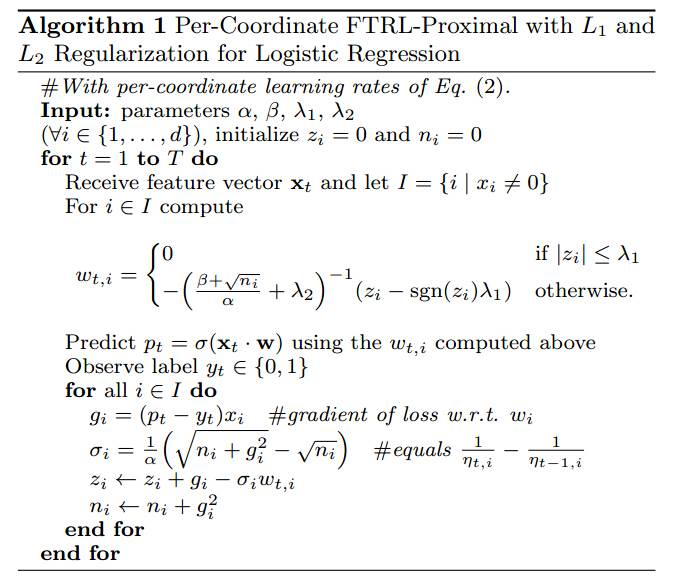但其实FTRL是一个online learning的框架，能解决的问题绝不仅仅是LR，已经成了一个通用的优化算子，比如TensorFlow的optimizer中都包含了FTRL。我们只要把截图中的伪代码修改，$p_t$的计算改为 $\hat{y}(x|\Theta)$，对于每一轮的特征向量$x$的每一维非0特征$x_i$，都要相应的更新模型参数$w_0,w_i,v_{i,1},…,v_{i,k}$，更新公式不变和截图一致，梯度$g$的计算即为损失函数对每个参数的偏导，前面已经给出。$\sigma,z,n$的更新公式不变。伪代码如下：

Algorithm: alphaFM

$Input:paramters\,\alpha^w,\alpha^v,\beta^w,\beta^v,\lambda_1^w,\lambda_1^v,\lambda_2^w,\lambda_2^v,\sigma$
$Init:w_0=0;n_0^w=0;z_0^w=0;$
$Init:\forall i,\forall f,w_i=0;n_i^w=0;z_i^w=0;v_{i,f}\sim N(0,\sigma);n_{i,f}^v=0;z_{i,f}^v=0;$
$for\,t=1\,to\,T,do$
$\qquad Receive\,feature\,vector\,x\,and\,let\,I=\{i|x_i\neq0\}$
$$w_0= \begin{cases} 0 & if\,\,|z_0^w|\le\lambda_1^w \\ -\left(\frac{\beta^w+\sqrt{n_0^w}}{\alpha^w}+\lambda_2^w\right)^{-1}(z_0^w-sgn(z_0^w)\lambda_1^w) & otherwise. \\ \end{cases}$$
$\qquad for\,i\in I,compute$
$$w_i= \begin{cases} 0 & if\,\,|z_i^w|\le\lambda_1^w \\ -\left(\frac{\beta^w+\sqrt{n_i^w}}{\alpha^w}+\lambda_2^w\right)^{-1}(z_i^w-sgn(z_i^w)\lambda_1^w) & otherwise. \\ \end{cases}$$
$\qquad \qquad for\,f=1\,to\,k,compute$
$$v_{i,f}= \begin{cases} 0 & if\,\,|z_{i,f}^v|\le\lambda_1^v \\ -\left(\frac{\beta^v+\sqrt{n_{i,f}^v}}{\alpha^v}+\lambda_2^v\right)^{-1}(z_{i,f}^v-sgn(z_{i,f}^v)\lambda_1^v) & otherwise. \\ \end{cases}$$
$\qquad \qquad end\,for$
$\qquad end\,for$
$\qquad Compute\,\hat{y}(x|\Theta)$
$\qquad Observe\,label\,y\in\{-1,1\}$
$\qquad compute\,g_0^w$
$\qquad \sigma_0^w=\frac{1}{\alpha^w}(\sqrt{n_0^w+(g_0^w)^2}-\sqrt{n_0^w})$
$\qquad z_0^w\leftarrow z_0^w+g_0^w-\sigma_0^ww_0$
$\qquad n_0^w\leftarrow n_0^w+(g_0^w)^2$
$\qquad for\,i\in I,do$
$\qquad \qquad compute\,g_i^w$
$\qquad \qquad \sigma_i^w=\frac{1}{\alpha^w}(\sqrt{n_i^w+(g_i^w)^2}-\sqrt{n_i^w})$
$\qquad \qquad z_i^w\leftarrow z_i^w+g_i^w-\sigma_i^ww_i$
$\qquad \qquad n_i^w\leftarrow n_i^w+(g_i^w)^2$
$\qquad \qquad for\,f=1\,to\,k,do$
$\qquad \qquad \qquad compute\,g_{i,f}^v$
$\qquad \qquad \qquad \sigma_{i,f}^v=\frac{1}{\alpha^v}(\sqrt{n_{i,f}^v+(g_{i,f}^v)^2}-\sqrt{n_{i,f}^v})$
$\qquad \qquad \qquad z_{i,f}^v\leftarrow z_{i,f}^v+g_{i,f}^v-\sigma_{i,f}^vv_{i,f}$
$\qquad \qquad \qquad n_{i,f}^v\leftarrow n_{i,f}^v+(g_{i,f}^v)^2$
$\qquad \qquad end\,for$
$\qquad end\,for$
$end\,for$

## 多分类问题

Softmax模型是LR在多分类上的推广，具体介绍戳这里。大致就是如果有$c$个类别，则模型参数为$c$个向量：$\Theta=\{w_1,w_2,…,w_c\}$，其中任意$w_i\in R^n$。

样本$x$属于类别$i$的概率：
$$P(y=i|x,\Theta)=\frac{e^{w_i^Tx}}{\sum_{j=1}^ce^{w_j^Tx}}$$
FM解决多分类的方法同样是将线性部分$w^Tx$替换成复杂的 $\hat{y}(x|\Theta)$，不过不再是一个 $\hat{y}$，而是每一类别对应一个，共$c$个：$\hat{y}_1(x|\Theta),…,\hat{y}_c(x|\Theta)$

样本$x$属于类别$i$的概率也变成：
$$P(y=i|x,\Theta)=\frac{e^{\hat{y}_i(x|\Theta)}}{\sum_{j=1}^ce^{\hat{y}_j(x|\Theta)}}$$
模型参数一共$c$组， $\Theta=\{\Theta_1,…,\Theta_c\}$，其中类别$i$对应一组参数 $\Theta_i=\{w_0^i,w_1^i,…,w_n^i,v_{1,1}^i,…,v_{n,k}^i\}$

我们定义一个示性函数 $1\{\cdot\}$，大括号中表达式为真则值为1，表达式为假则值为0。这样就可以写出最优化问题：
$$\mathop{\arg\max}_{\Theta}\prod_{(x,y)\in S}\prod_{i=1}^cP(y=i|x,\Theta)^{1\{y=i\}}\\ =\mathop{\arg\min}_{\Theta}-\sum_{(x,y)\in S}\sum_{i=1}^c1\{y=i\}\ln P(y=i|x,\Theta)$$
每条样本 $(x,y)$ 的损失函数：
$$l(\Theta|x,y)=-\sum_{i=1}^c1\{y=i\}\ln P(y=i|x,\Theta)\\ =-\sum_{i=1}^c1\{y=i\}\ln \frac{e^{\hat{y}_i(x|\Theta)}}{\sum_{j=1}^ce^{\hat{y}_j(x|\Theta)}}\\ =\sum_{i=1}^c1\{y=i\}(\ln\sum_{j=1}^ce^{\hat{y}_j(x|\Theta)}-\hat{y}_i(x|\Theta))\\ =\ln\sum_{j=1}^ce^{\hat{y}_j(x|\Theta)}-\sum_{i=1}^c1\{y=i\}\hat{y}_i(x|\Theta)$$
梯度：
$$\nabla_{\Theta_i}l(\Theta|x,y)=\frac{\partial l}{\partial\hat{y}_i}\nabla_{\Theta_i}\hat{y}_i(x|\Theta)$$
而
$$\frac{\partial l}{\partial\hat{y}_i}=\frac{e^{\hat{y}_i(x|\Theta)}}{\sum_{j=1}^ce^{\hat{y}_j(x|\Theta)}}-1\{y=i\}\\ =P(y=i|x,\Theta)-1\{y=i\}$$
所以有
$$\nabla_{\Theta_i}l(\Theta|x,y)=(P(y=i|x,\Theta)-1\{y=i\})\nabla_{\Theta_i}\hat{y}_i(x|\Theta)$$
$\nabla_{\Theta_i}\hat{y}_i(x|\Theta)$ 即求 $\hat{y}_i$ 对 $\Theta_i$ 中所有参数 $\{w_0^i,w_1^i,…,w_n^i,v_{1,1}^i,…,v_{n,k}^i\}$ 的偏导，这在二分类中我们已经给出。

最后，仍然是套用FTRL的框架，只是每条样本更新的参数更多，不再细说，详见代码。

# CF的ALS算法推导

在上一篇中介绍了矩阵微分，现在就来牛刀小试一下。早些时候子龙问过我 Collaborative Filtering for Implicit Feedback Datasets这篇论文里的公式推导，在这里重新解一遍。
论文里给出的目标函数为：
$$\min_{x_*,y_*}\left\{\sum_{u,i}c_{ui}(p_{ui}-x_u^Ty_i)^2+\lambda(\sum_u||x_u||^2+\sum_i||y_i||^2)\right\}\,\,\,\,(1)$$
模型的来历不是今天的重点，简单描述一下各个变量的含义，其中$u$代表user，$i$代表item，$x_u\in R^f$，$y_i\in R^f$，未出现的变量 $r_{ui}$ 表示user对item的“消费”度量，
$$p_{ui}= \begin{cases} 1 & r_{ui}>0 \\ 0 & r_{ui}=0 \\ \end{cases}$$

假设user的数目为$m$，item的数目为$n$，则目标函数包含的项大约有$m\cdot n$，通常数量非常巨大，一些常见的优化算法比如SGD不再适合，论文中采用了ALS（alternating-least-squares）算法，这是一种迭代的方法，第一步先固定所有的$y_i$，求解最优的$x_u$；第二步固定所有的$x_u$，求解最优的$y_i$，依次迭代。
我们先看第一步，当固定所有$y_i$后，(1)式转化为
$$\min_{x_*}\left\{\sum_{u,i}c_{ui}(p_{ui}-x_u^Ty_i)^2+\lambda\sum_u||x_u||^2\right\}\\ =\sum_u\min_{x_u}\left\{\sum_{i}c_{ui}(p_{ui}-x_u^Ty_i)^2+\lambda||x_u||^2\right\}\\ =\sum_u\min_{x_u}L_u(x_u)$$

对每个$u$，我们定义一个$n\times n$的对角矩阵$C^u$，其中$C_{ii}^u=c_{ui}$，定义向量$p(u)\in R^n$包含所有的$p_{ui}$。激动人心的时刻来到了，我们开始推导函数$L_u(x_u)$ 对$x_u$的微分
$$L_u(x_u)=\sum_{i}c_{ui}(p_{ui}-x_u^Ty_i)^2+\lambda||x_u||^2\\ = \sum_{i}c_{ui}(y_i^Tx_u-p_{ui})^2+\lambda x_u^Tx_u\\ dL_u=\sum_{i}2c_{ui}(x_u^Ty_i-p_{ui})y_i^Tdx_u+2\lambda x_u^Tdx_u\\ =2\left(\sum_{i}c_{ui}x_u^Ty_iy_i^T-\sum_{i}c_{ui}p_{ui}y_i^T+\lambda x_u^T\right)dx_u\\$$
$L_u(x_u)$ 取极值，须$dL_u=0$，有
$$\sum_{i}c_{ui}x_u^Ty_iy_i^T-\sum_{i}c_{ui}p_{ui}y_i^T+\lambda x_u^T=0\\ \Rightarrow \sum_{i}c_{ui}y_iy_i^Tx_u+\lambda Ix_u=\sum_{i}c_{ui}p_{ui}y_i\\ \Rightarrow \left(\sum_{i}c_{ui}y_iy_i^T+\lambda I\right)x_u=\sum_{i}c_{ui}p_{ui}y_i\,\,\,\,(2)$$
按论文中的符号定义，设$Y^T=(y_1,\cdots,y_n)$，有
$$\sum_{i}c_{ui}y_iy_i^T=(c_{u1}y_1,\cdots,c_{un}y_n) \left(\begin{array}{c} y_1^T \\ \vdots \\ y_n^T \\ \end{array}\right)\\ =(y_1,\cdots,y_n) \left(\begin{array}{ccc} c_{u1} & & \\ & \ddots & \\ & & c_{un} \\ \end{array}\right) \left(\begin{array}{c} y_1^T \\ \vdots \\ y_n^T \\ \end{array}\right)\\ =Y^TC^uY$$
类似地，有 $\sum_{i}c_{ui}p_{ui}y_i=Y^TC^up(u)$，因此
$$(2)\Rightarrow (Y^TC^uY+\lambda I)x_u=Y^TC^up(u)\\ \Rightarrow x_u=(Y^TC^uY+\lambda I)^{-1}Y^TC^up(u)$$

# 矩阵微分(Matrix Differential)

在最优化问题中，经常涉及到导数或梯度的计算，比如 $\nabla(x’Ax)$，虽然自己最终也能推导出来等于 $(A+A’)x$，但总感觉在这块缺少系统的知识，有时还会想向量函数的导数又如何计算，矩阵函数呢？在网上搜了很久，似乎这块的知识点一直比较混乱，wikipedia的Matrix calculus词条也写得不好，甚至有人质疑。大约一年前又去中关村图书大厦遍寻矩阵相关的书籍，只找到清华出的一本《矩阵分析与应用》涉及到矩阵微分，手机拍了几十张照片回来细读仍不甚满意。无意间却从网上找到了这本书：Matrix Differential Calculus with Applications in Statistics and Econometrics，正是我想要的！（其实清华那本和wikipedia都提到了这本书，只是当时没注意。）书甚厚，博大精深，我把感兴趣的部分边看边做笔记，唯纸薄字陋难存久，便想着不如写成blog，可惜本是懒惰之人一拖便是一年，直到最近失意百无聊赖，终于完成。文章憎命达，诚如是。

## 1. 基础概念

向量$x$的范数(norm)定义为：
$$||x||=(x’x)^{1/2}$$

假设$a$为$n \times 1$向量，$A$为$n \times n$矩阵，$B$为$n \times m$矩阵，则$a’x$称为向量$x$的线性型，$x’Ax$称为$x$的二次型，$x’By$称为$x$和$y$的双线性型。
不失一般性，我们假定$A$为对称阵，因为我们总可以用 $(A+A’)/2$ 来代替，推导如下：
$$x’(A+A’)x=x’Ax+x’A’x=x’Ax+(x’A’x)’=x’Ax+x’Ax=2x’Ax$$
我们说，$A$是正定的，如果对所有$x\ne0$，有$x’Ax>0$；$A$是半正定的，如果对所有$x$，有$x’Ax\ge0$。负定的定义类似，不再赘述。
易证 $BB’$ 和 $B’B$ 都是半正定的，因为 $x’B’Bx=(Bx)’Bx\ge0$

方阵的迹(trace)定义为：

$$trA=\sum_{i=1}^na_{ii}$$

矩阵的范数定义为：

$$||A||=(trA’A)^{1/2}$$

矩阵的直积：
$A$为$m\times n$矩阵，$B$为$p\times q$矩阵，则$A\otimes B$为$mp\times nq$的矩阵
$$A\otimes B= \left(\begin{array}{ccc} a_{11}B & \cdots & a_{1n}B \\ \vdots & & \vdots \\ a_{m1}B & \cdots & a_{mn}B \\ \end{array}\right)$$

VEC算子：
设$A$为$m\times n$矩阵，$a_i$为其第$i$列，则$vec\,A$为$mn\times 1$向量
$$vec\,A= \left(\begin{array}{c} a_1 \\ a_2 \\ \vdots \\ a_n \end{array}\right)$$
几个相关公式:
$$vec\,a’=vec\,a=a$$
$$vec\,ab’=b\otimes a$$
$$(vec\,A)’vec\,B=tr\,A’B$$
$$vec\,ABC=(C’\otimes A)vec\,B$$
$$vec\,AB=(B’\otimes I_m)vec\,A=(B’\otimes A)vec\,I_n=(I_q\otimes A)vec\,B,\,\,\,\,A:m\times n,B:n\times q$$

## 2. 微分(differential)的定义

### 2.1 标量函数的微分

对于一维的情况，$\phi(x):R\rightarrow R$
$$\phi(c+u)=\phi(c)+u\phi’(c)+r_c(u)$$
$$\lim_{u\rightarrow 0}\frac{r_c(u)}{u}=0$$
定义 $\phi$ 在$c$点基于增量$u$的一阶微分为
$$d\phi(c;u)=u\phi’(c)$$

### 2.2 向量函数的微分

设函数$f:S\rightarrow R^m$，$S\subset R^n$，$c$是$S$的一个内点(interior point)，$B(c;r)$ 是$S$中$c$点的一个邻域(n-ball)，$u$是$R^n$中的一点，满足 $||u||<r$，因此有$c+u\in B(c;r)$，如果存在一个$m\times n$实矩阵$A$，满足
$$f(c+u)=f(c)+A(c)u+r_c(u)$$

$$\lim_{u\rightarrow0}\frac{r_c(u)}{||u||}=0$$

$$df(c;u)=A(c)u$$

### 2.3 向量函数的偏导数

令$f=(f_1,f_2,\cdots,f_m)$，$t\in R$，如果极限
$$\lim_{t\rightarrow0}\frac{f_i(c+te_j)-f_i(c)}{t}$$

如果$f$在$c$点可微，则所有的偏导数$D_jf_i(c)$ 存在。反过来不一定成立。

如果$f$在$c$点可微，那么存在矩阵$A(c)$，
$$f(c+u)=f(c)+A(c)u+r_c(u)$$

$A(c)$ 的每一项$a_{ij}(c)$ 其实就是相应的偏导数$D_jf_i(c)$，即
$$A(c)=Df(c)$$

$Df(c)$ 的转置称为$f$在$c$点的梯度，用 $\nabla f(c)$ 表示，即
$$\nabla f(c)=(Df(c))’$$
当向量函数$f:S\rightarrow R^m$ 退化成标量函数 $\phi:S\rightarrow R$ 时，雅可比矩阵退化成$1\times n$行向量$D\phi(c)$，梯度退化成$n\times 1$列向量 $\nabla\phi(c)$。

### 2.5 矩阵函数的微分

现在终于轮到矩阵函数出场了，令 $F:S\rightarrow R^{m\times p}$，其中 $S\subset R^{n\times q}$。$C$是$S$的内点，且令 $B(C;r)=\{X:X\in R^{n\times q},||X-C||<r\}$，这里 $||X||=(trX’X)^{1/2}$。
设点$U$是$R^{n\times q}$ 内一点，满足 $||U||<r$，因此有 $C+U\in B(C;r)$。如果存在一个 $mp\times nq$ 的矩阵 $A$，有
$$vec\,F(C+U)=vec\,F(C)+A(C)vec\,U+vec\,R_C(U)$$

$$\lim_{U\rightarrow0}\frac{R_C(U)}{||U||}=0$$

$m\times p$ 矩阵 $dF(C;U)$ 由下式定义
$$vec\,dF(C;U)=A(C)vec\,U$$

可以看到，这里的做法就是将矩阵函数化为向量函数来处理。对于每个矩阵函数 $F$（自变量和函数值均为矩阵），

$$f(vec\,X)=vec\,F(X)$$

$$vec\,dF(C;U)=df(vec\,C;vec\,U)$$

$$DF(C)=Df(vec\,C)$$

当 $F$ 在 $C$ 点可微，有 $DF(C)=A(C)$

设 $U$ 和 $V$ 是矩阵函数，$A$ 是矩阵常量，有
$$dA=0$$
$$d(\alpha U)=\alpha dU$$
$$d(U+V)=dU+dV$$
$$d(U-V)=dU-dV$$
$$d(UV)=(dU)V+UdV$$
$$dU’=(dU)’$$
$$d\,vec\,U=vec\,dU$$
$$d\,tr\,U=tr\,dU$$

### 2.6 链式法则

同最简单的标量自变量的标量函数一样，链式法则同样成立。
设函数$f:S\rightarrow R^m$，$S\subset R^n$，在 $c$ 点可微。$f(x)\in T$，设函数$g:T\rightarrow R^p$在 $b=f(c)$ 点可微。定义复合函数$h:S\rightarrow R^p$ 如下：
$$h(x)=g(f(x))$$

$$Dh(c)=(Dg(b))(Df(c))$$
$$dh(c;u)=(Dg(b))(Df(c))u=(Dg(b))df(c;u)=dg(b;df(c;u))$$
对矩阵函数类似，省略掉函数定义，直接给出公式：
$$H(X)=G(F(X))$$
$$DH(C)=(DG(B))(DF(C))$$
$$dH(C;U)=dG(B;dF(C;U))$$
我们还可以简写微分符号：
$$y=g(t)$$
$$dy=dg(t;dt)$$
$$t=f(x)$$
$$y=g(f(x))\equiv h(x)$$
$$dy=dh(x;dx)=dg(f(x);df(x;dx))=dg(t;dt)$$
我们甚至可以不用$y$直接用$g$本身来简写：
$$dg=dg(t;dt)$$
举例：
$$y=\phi(x)=e^{x’x}\\ dy=de^{x’x}=e^{x’x}(dx’x)=e^{x’x}((dx)’x+x’dx)=(2e^{x’x}x’)dx$$
$$z=\phi(\beta)=(y-X\beta)’(y-X\beta)$$

$$dz=de’e=2e’de=2e’d(y-X\beta)\\ =-2e’Xd\beta=-2(y-X\beta)’Xd\beta$$

## 3. 雅可比矩阵(Jacobian matrix)

重新梳理一下雅克比矩阵的求法，基本思路如下：
给定一个矩阵函数$F(X)$
(1)计算$F(X)$ 的微分
(2)向量化得到$d\,vec\,F(X)=A(X)d\,vec\,X$
(3)得到雅克比矩阵$DF(X)=A(X)$

我们再次明确一下自变量和函数的符号，见下表
$$\begin{array}{cccc} \hline & Scalar & Vector & Matrix \\ & variable & variable & variable \\ \hline Scalar\,function & \phi(\xi) & \phi(x) & \phi(X) \\ Vector\,function & f(\xi) & f(x) & f(X) \\ Matrix\,function & F(\xi) & F(x) & F(X) \\ \hline \end{array}$$

雅克比矩阵本质上就是要解决如何排列 $F(X)$ 的所有偏导数 $\partial f_{st}(X)/\partial x_{ij}$ 的问题。
先给出一些符号定义：
$\phi$为标量函数，$X=(x_{ij})$ 为 $n\times q$ 矩阵，$F=(f_{st})$ 为 $m\times p$ 矩阵
$$\frac{\partial\phi(X)}{\partial X}= \left(\begin{array}{ccc} \partial\phi/\partial x_{11} & \cdots & \partial\phi/\partial x_{1q} \\ \vdots & & \vdots \\ \partial\phi/\partial x_{n1} & \cdots & \partial\phi/\partial x_{nq} \\ \end{array}\right)$$

$$\frac{\partial F(X)}{\partial X}= \left(\begin{array}{ccc} \partial f_{11}/\partial X & \cdots & \partial f_{1p}/\partial X \\ \vdots & & \vdots \\ \partial f_{m1}/\partial X & \cdots & \partial f_{mp}/\partial X \\ \end{array}\right)$$

特别地，$\phi$为标量函数，$\partial\phi/\partial x$是列向量，$\partial\phi/\partial x’$ 是行向量；$m\times1$的向量函数$f(x)$，$x$为$n\times1$向量，可以有四种排列：$\partial f/\partial x’$（$m\times n$矩阵），$\partial f’/\partial x$（$n\times m$矩阵），$\partial f/\partial x$（$mn\times 1$矩阵），$\partial f’/\partial x’$（$1\times mn$矩阵）。
下面给出雅克比矩阵的符号定义：
$$D\phi(x)=(D_1\phi(x),\dots,D_n(x))=\frac{\partial\phi(x)}{\partial x’}$$
$$Df(x)=\frac{\partial f(x)}{\partial x’}$$
$$DF(X)=\frac{\partial\,vec\,F(X)}{\partial(vec\,X)’}$$
可以对比一下，$DF(X)$ 是 $mp\times nq$，而 $\partial F(X)/\partial X$ 是$mn\times pq$。
举几个例子，$F(X)=AXB$，则
$$dF(X)=A(dX)B$$
$$d\,vec\,F(X)=(B’\otimes A)d\,vec\,X$$
因此有
$$DF(X)=B’\otimes A$$
令 $\phi(x)=x’Ax$，则
$$d\phi(x)=d(x’Ax)=(dx)’Ax+x’Adx\\ =((dx)’Ax)’+x’Ax=x’A’dx+x’Adx\\ =x’(A+A’)dx$$
因此有
$$D\phi(x)=x’(A+A’)$$